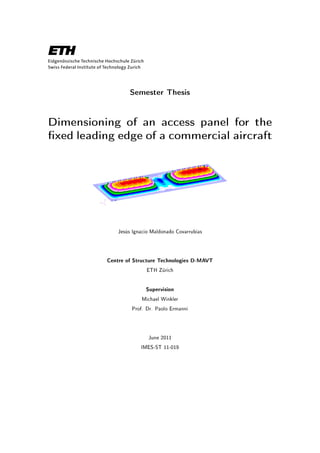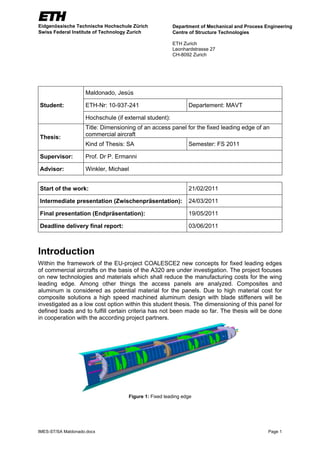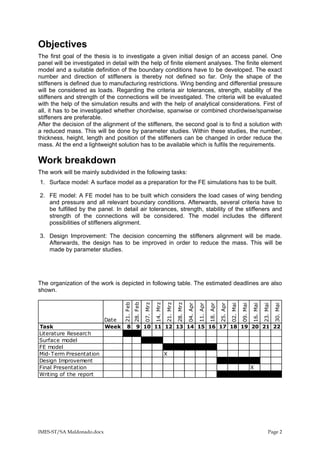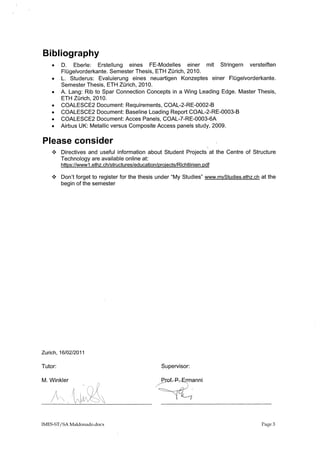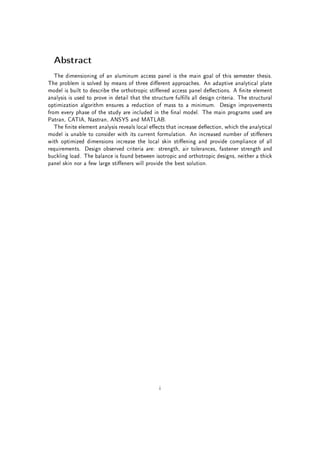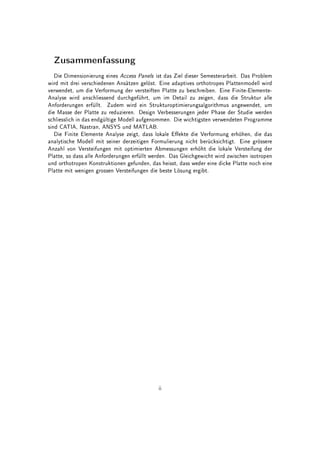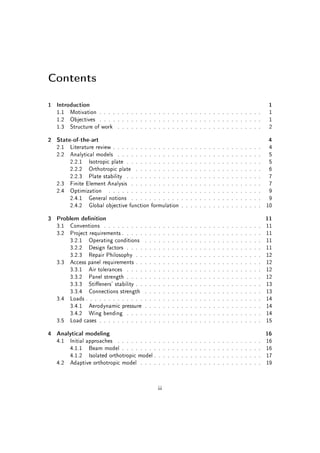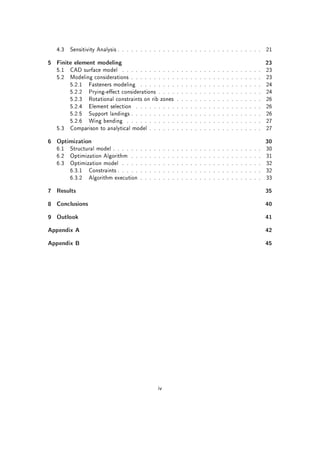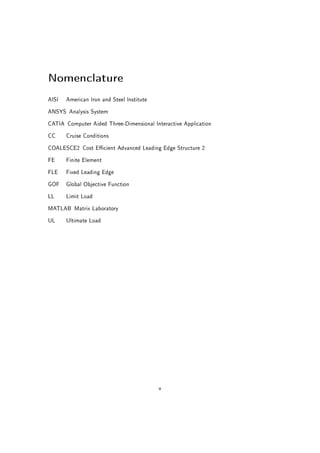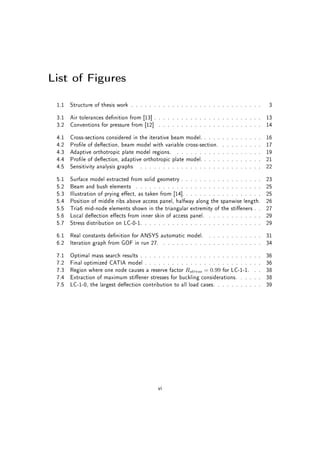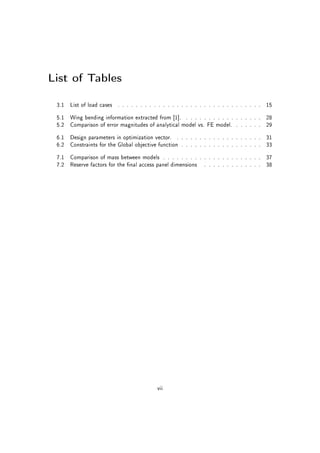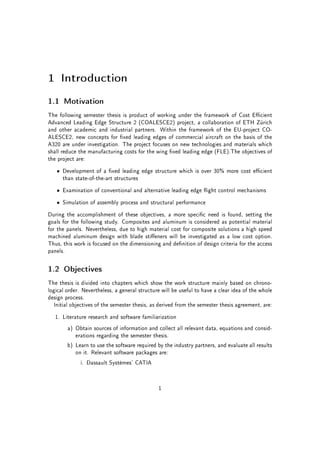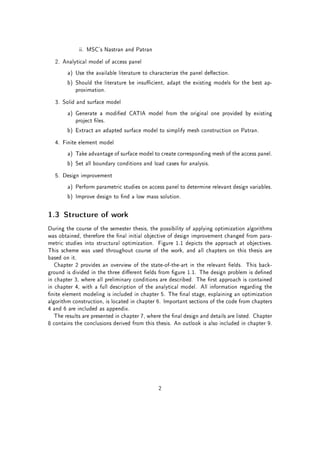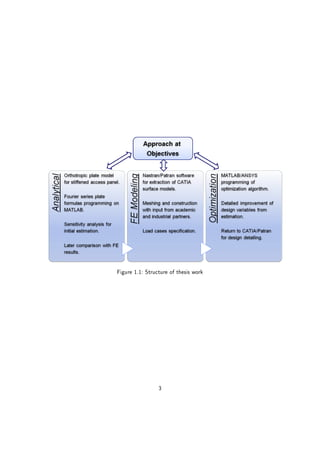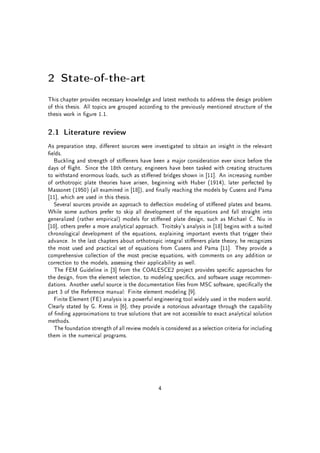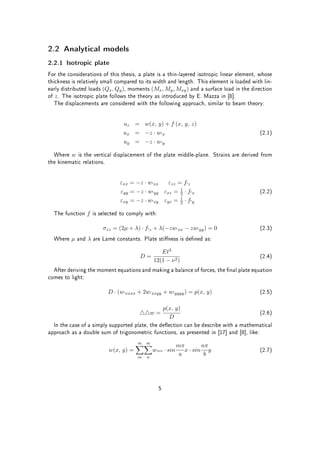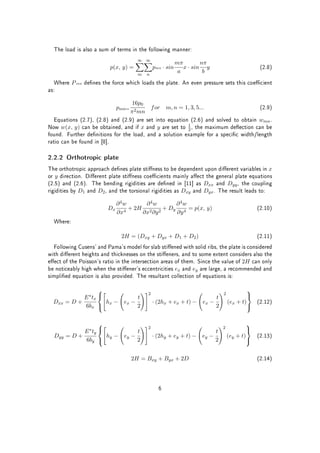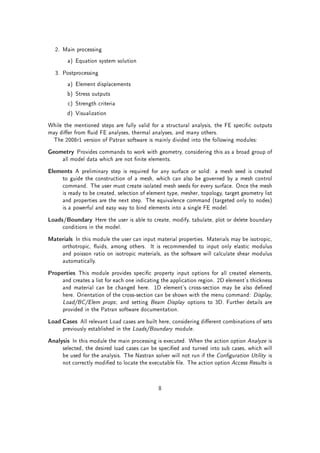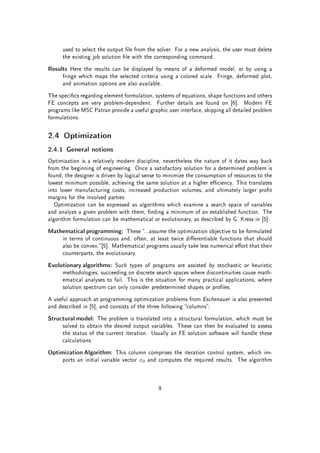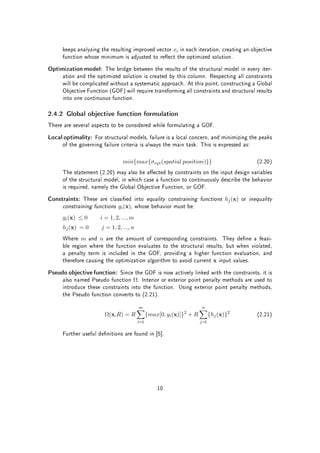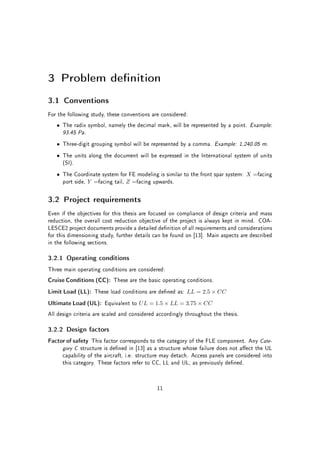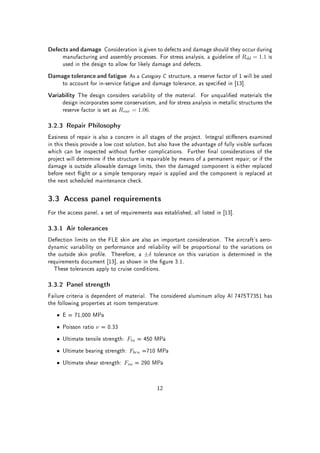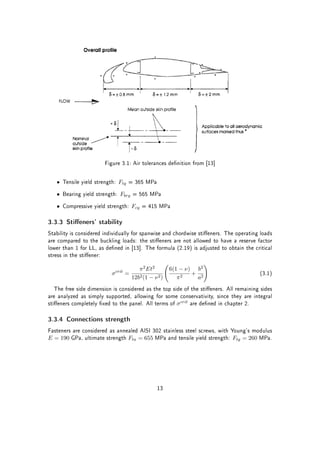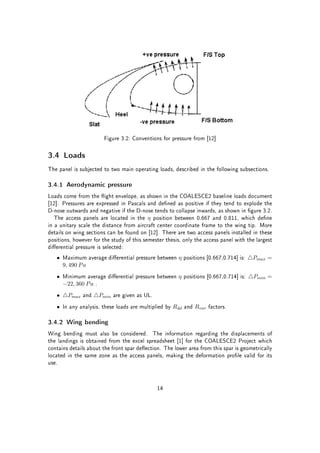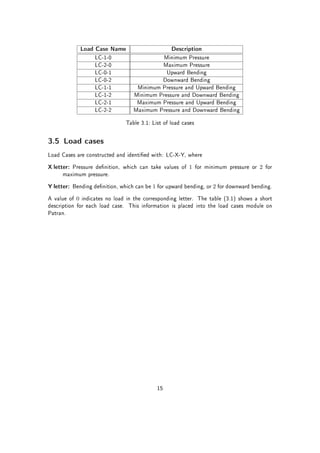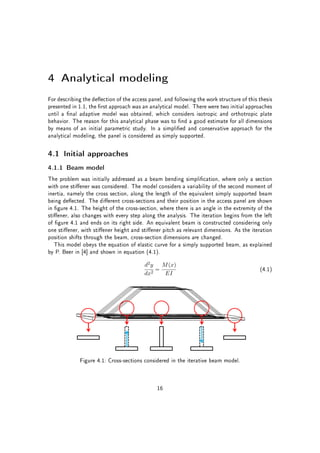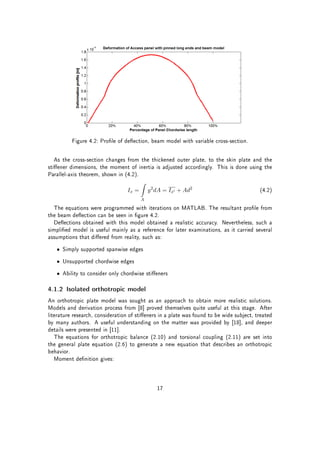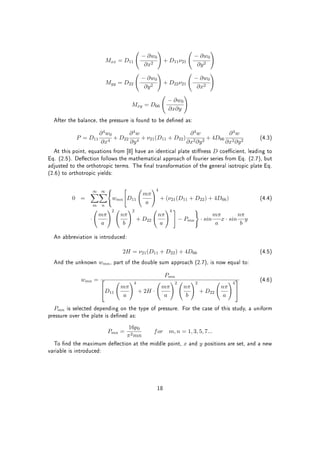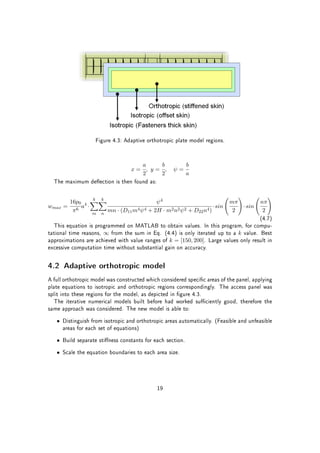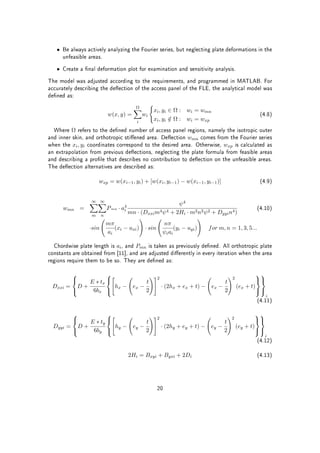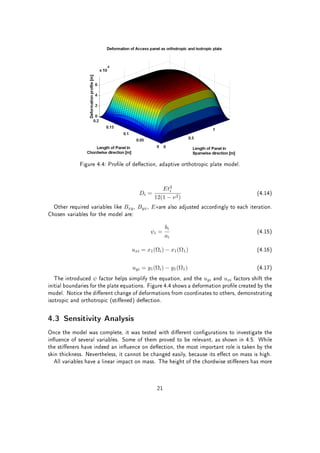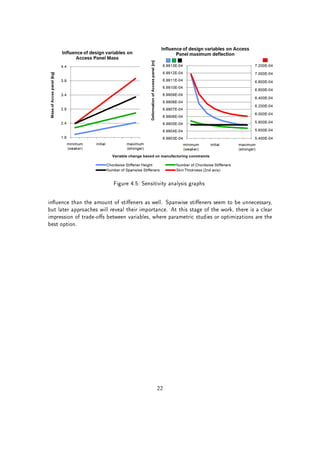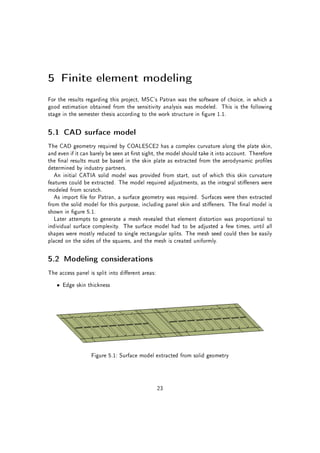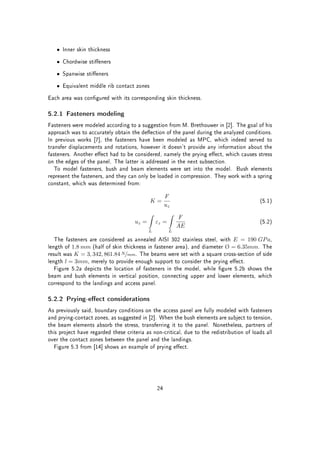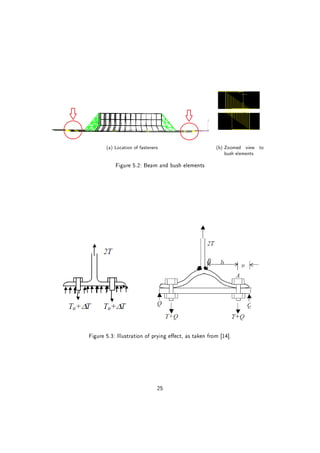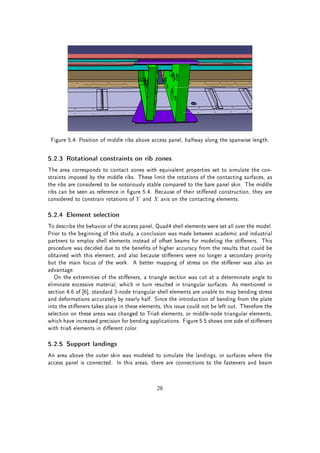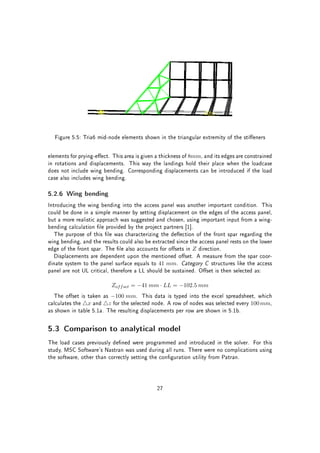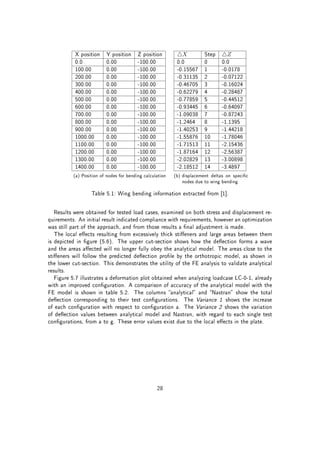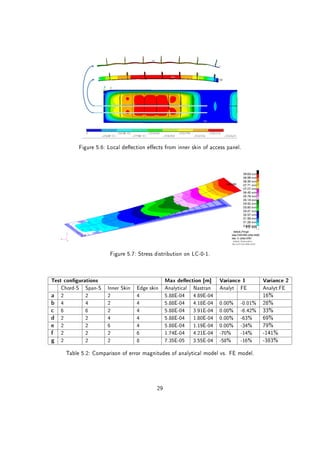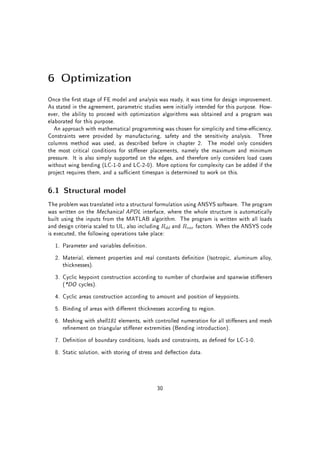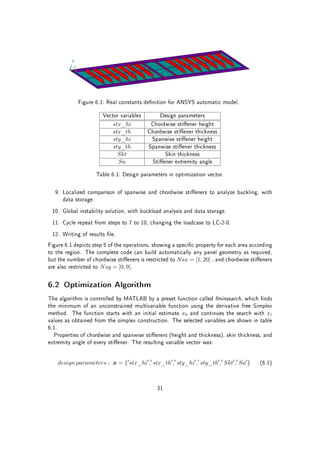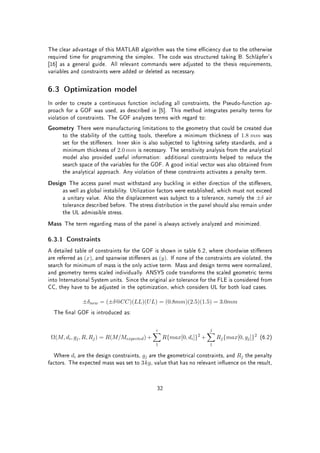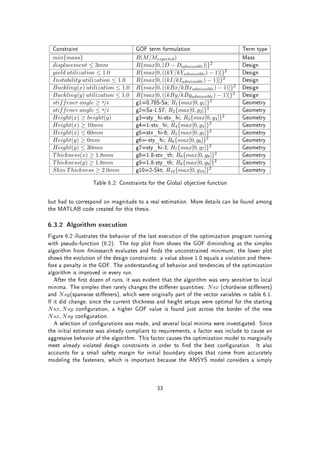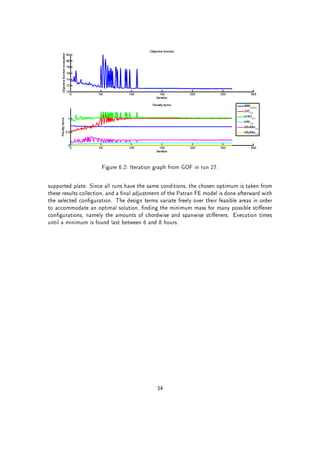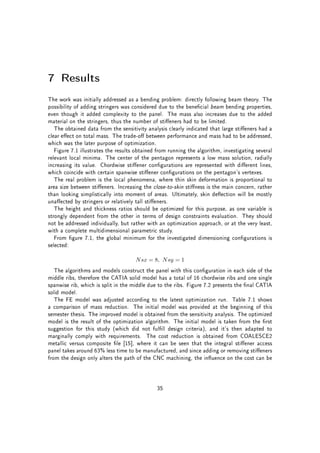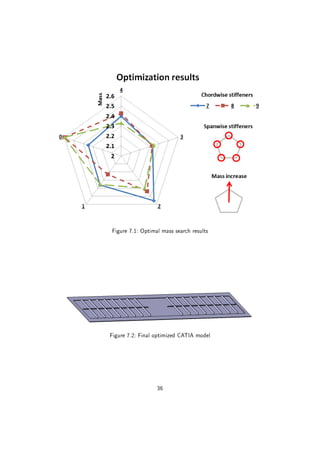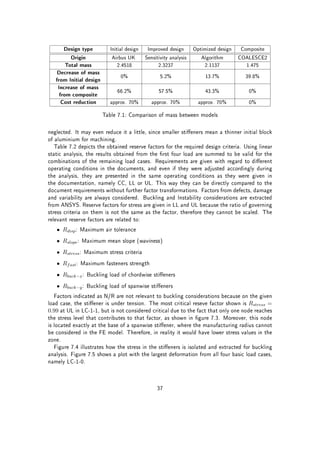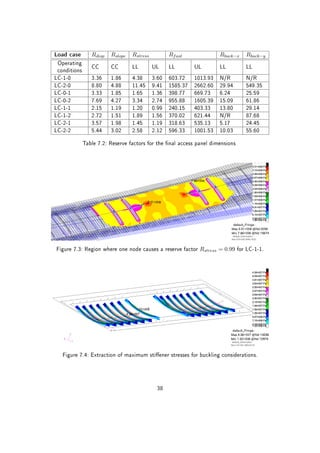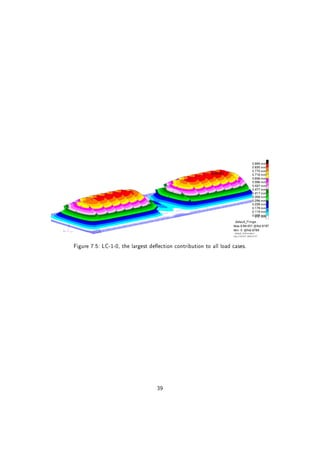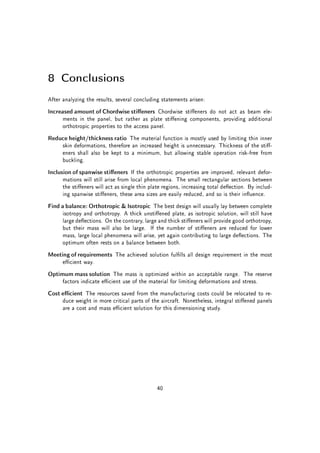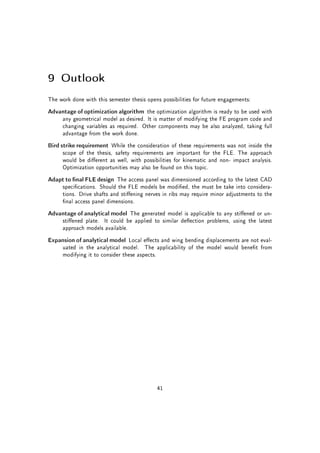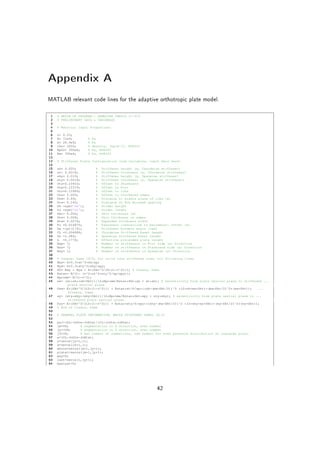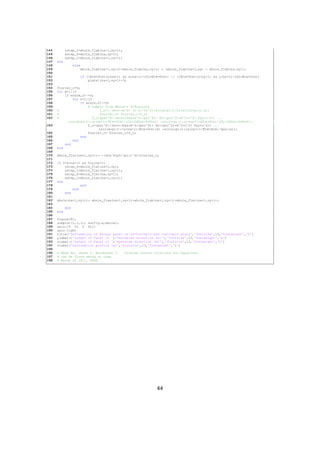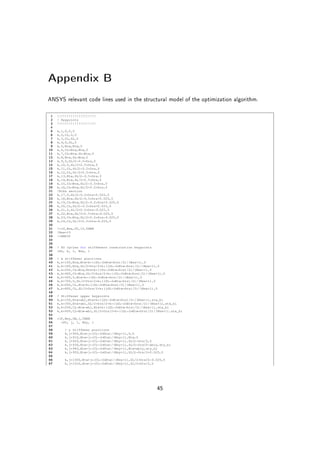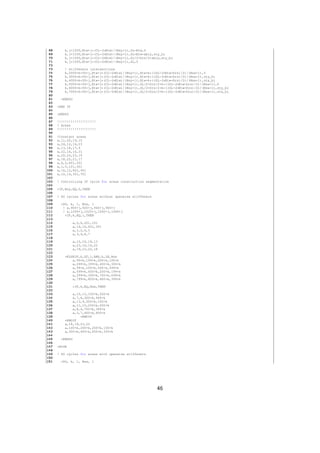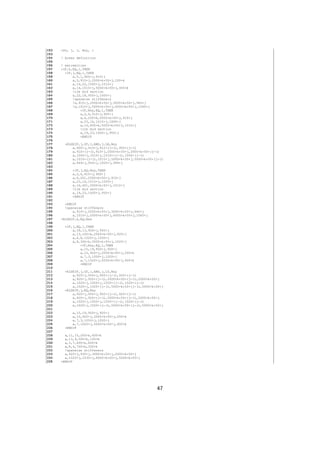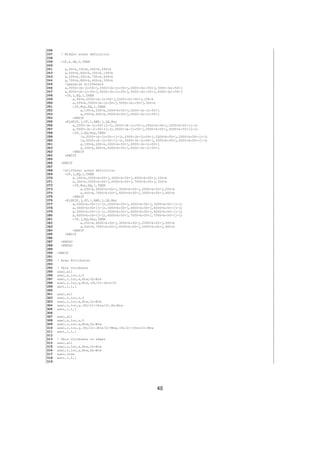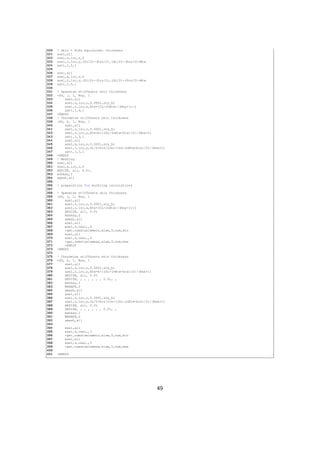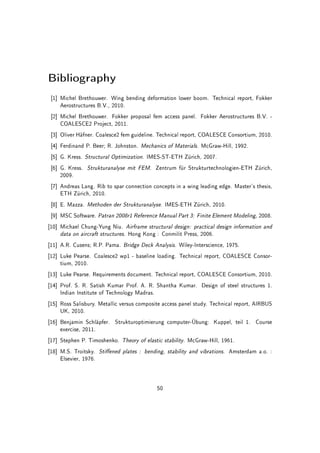Próximo SlideShare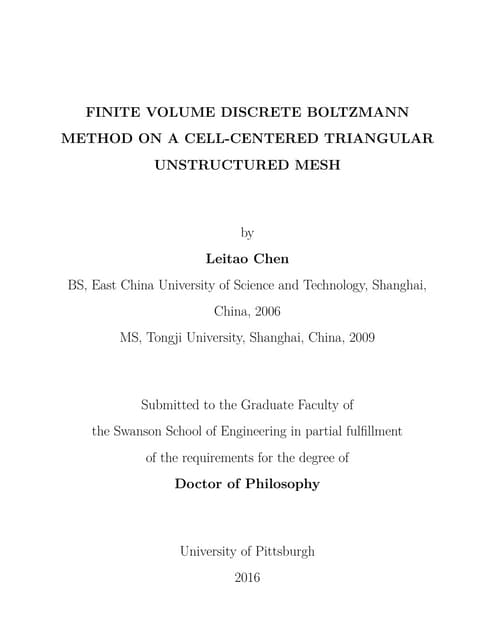LChen_diss_Pitt_FVDBM
Cargando en ... 3
1 de 61

1. Semester Thesis Dimensioning of an access panel for the xed leading edge of a commercial aircraft Jesús Ignacio Maldonado Covarrubias Centre of Structure Technologies D-MAVT ETH Zürich Supervision Michael Winkler Prof. Dr. Paolo Ermanni June 2011 IMES-ST 11-019
3. IMES-ST/SA Maldonado.docx Page 2 Objectives The first goal of the thesis is to investigate a given initial design of an access panel. One panel will be investigated in detail with the help of finite element analyses. The finite element model and a suitable definition of the boundary conditions have to be developed. The exact number and direction of stiffeners is thereby not defined so far. Only the shape of the stiffeners is defined due to manufacturing restrictions. Wing bending and differential pressure will be considered as loads. Regarding the criteria air tolerances, strength, stability of the stiffeners and strength of the connections will be investigated. The criteria will be evaluated with the help of the simulation results and with the help of analytical considerations. First of all, it has to be investigated whether chordwise, spanwise or combined chordwise/spanwise stiffeners are preferable. After the decision of the alignment of the stiffeners, the second goal is to find a solution with a reduced mass. This will be done by parameter studies. Within these studies, the number, thickness, height, length and position of the stiffeners can be changed in order reduce the mass. At the end a lightweight solution has to be available which is fulfils the requirements. Work breakdown The work will be mainly subdivided in the following tasks: 1. Surface model: A surface model as a preparation for the FE simulations has to be built. 2. FE model: A FE model has to be built which considers the load cases of wing bending and pressure and all relevant boundary conditions. Afterwards, several criteria have to be fulfilled by the panel. In detail air tolerances, strength, stability of the stiffeners and strength of the connections will be considered. The model includes the different possibilities of stiffeners alignment. 3. Design Improvement: The decision concerning the stiffeners alignment will be made. Afterwards, the design has to be improved in order to reduce the mass. This will be made by parameter studies. The organization of the work is depicted in following table. The estimated deadlines are also shown. Date 21.Feb 28.Feb 07.Mrz 14.Mrz 21.Mrz 28.Mrz 04.Apr 11.Apr 18.Apr 25.Apr 02.Mai 09.Mai 16.Mai 23.Mai 30.Mai Task Week 8 9 10 11 12 13 14 15 16 17 18 19 20 21 22 Literature Research Surface model FE model Mid-Term Presentation X Design Improvement Final Presentation X Writing of the report
4. Abstract The dimensioning of an aluminum access panel is the main goal of this semester thesis. The problem is solved by means of three dierent approaches. An adaptive analytical plate model is built to describe the orthotropic stiened access panel deections. A nite element analysis is used to prove in detail that the structure fullls all design criteria. The structural optimization algorithm ensures a reduction of mass to a minimum. Design improvements from every phase of the study are included in the nal model. The main programs used are Patran, CATIA, Nastran, ANSYS and MATLAB. The nite element analysis reveals local eects that increase deection, which the analytical model is unable to consider with its current formulation. An increased number of stieners with optimized dimensions increase the local skin stiening and provide compliance of all requirements. Design observed criteria are: strength, air tolerances, fastener strength and buckling load. The balance is found between isotropic and orthotropic designs, neither a thick panel skin nor a few large stieners will provide the best solution. i
5. Zusammenfassung Die Dimensionierung eines Access Panels ist das Ziel dieser Semesterarbeit. Das Problem wird mit drei verschiedenen Ansätzen gelöst. Eine adaptives orthotropes Plattenmodell wird verwendet, um die Verformung der versteiften Platte zu beschreiben. Eine Finite-Elemente- Analyse wird anschliessend durchgeführt, um im Detail zu zeigen, dass die Struktur alle Anforderungen erfüllt. Zudem wird ein Strukturoptimierungsalgorithmus angewendet, um die Masse der Platte zu reduzieren. Design Verbesserungen jeder Phase der Studie werden schliesslich in das endgültige Modell aufgenommen. Die wichtigsten verwendeten Programme sind CATIA, Nastran, ANSYS und MATLAB. Die Finite Elemente Analyse zeigt, dass lokale Eekte die Verformung erhöhen, die das analytische Modell mit seiner derzeitigen Formulierung nicht berücksichtigt. Eine grössere Anzahl von Versteifungen mit optimierten Abmessungen erhöht die lokale Versteifung der Platte, so dass alle Anforderungen erfüllt werden. Das Gleichgewicht wird zwischen isotropen und orthotropen Konstruktionen gefunden, das heisst, dass weder eine dicke Platte noch eine Platte mit wenigen grossen Versteifungen die beste Lösung ergibt. ii
6. Contents 1 Introduction 1 1.1 Motivation . . . . . . . . . . . . . . . . . . . . . . . . . . . . . . . . . . . . 1 1.2 Objectives . . . . . . . . . . . . . . . . . . . . . . . . . . . . . . . . . . . . 1 1.3 Structure of work . . . . . . . . . . . . . . . . . . . . . . . . . . . . . . . . 2 2 State-of-the-art 4 2.1 Literature review . . . . . . . . . . . . . . . . . . . . . . . . . . . . . . . . . 4 2.2 Analytical models . . . . . . . . . . . . . . . . . . . . . . . . . . . . . . . . 5 2.2.1 Isotropic plate . . . . . . . . . . . . . . . . . . . . . . . . . . . . . . 5 2.2.2 Orthotropic plate . . . . . . . . . . . . . . . . . . . . . . . . . . . . 6 2.2.3 Plate stability . . . . . . . . . . . . . . . . . . . . . . . . . . . . . . 7 2.3 Finite Element Analysis . . . . . . . . . . . . . . . . . . . . . . . . . . . . . 7 2.4 Optimization . . . . . . . . . . . . . . . . . . . . . . . . . . . . . . . . . . 9 2.4.1 General notions . . . . . . . . . . . . . . . . . . . . . . . . . . . . . 9 2.4.2 Global objective function formulation . . . . . . . . . . . . . . . . . . 10 3 Problem denition 11 3.1 Conventions . . . . . . . . . . . . . . . . . . . . . . . . . . . . . . . . . . . 11 3.2 Project requirements . . . . . . . . . . . . . . . . . . . . . . . . . . . . . . . 11 3.2.1 Operating conditions . . . . . . . . . . . . . . . . . . . . . . . . . . 11 3.2.2 Design factors . . . . . . . . . . . . . . . . . . . . . . . . . . . . . . 11 3.2.3 Repair Philosophy . . . . . . . . . . . . . . . . . . . . . . . . . . . . 12 3.3 Access panel requirements . . . . . . . . . . . . . . . . . . . . . . . . . . . . 12 3.3.1 Air tolerances . . . . . . . . . . . . . . . . . . . . . . . . . . . . . . 12 3.3.2 Panel strength . . . . . . . . . . . . . . . . . . . . . . . . . . . . . . 12 3.3.3 Stieners' stability . . . . . . . . . . . . . . . . . . . . . . . . . . . . 13 3.3.4 Connections strength . . . . . . . . . . . . . . . . . . . . . . . . . . 13 3.4 Loads . . . . . . . . . . . . . . . . . . . . . . . . . . . . . . . . . . . . . . . 14 3.4.1 Aerodynamic pressure . . . . . . . . . . . . . . . . . . . . . . . . . . 14 3.4.2 Wing bending . . . . . . . . . . . . . . . . . . . . . . . . . . . . . . 14 3.5 Load cases . . . . . . . . . . . . . . . . . . . . . . . . . . . . . . . . . . . . 15 4 Analytical modeling 16 4.1 Initial approaches . . . . . . . . . . . . . . . . . . . . . . . . . . . . . . . . 16 4.1.1 Beam model . . . . . . . . . . . . . . . . . . . . . . . . . . . . . . . 16 4.1.2 Isolated orthotropic model . . . . . . . . . . . . . . . . . . . . . . . . 17 4.2 Adaptive orthotropic model . . . . . . . . . . . . . . . . . . . . . . . . . . . 19 iii
7. 4.3 Sensitivity Analysis . . . . . . . . . . . . . . . . . . . . . . . . . . . . . . . . 21 5 Finite element modeling 23 5.1 CAD surface model . . . . . . . . . . . . . . . . . . . . . . . . . . . . . . . 23 5.2 Modeling considerations . . . . . . . . . . . . . . . . . . . . . . . . . . . . . 23 5.2.1 Fasteners modeling . . . . . . . . . . . . . . . . . . . . . . . . . . . 24 5.2.2 Prying-eect considerations . . . . . . . . . . . . . . . . . . . . . . . 24 5.2.3 Rotational constraints on rib zones . . . . . . . . . . . . . . . . . . . 26 5.2.4 Element selection . . . . . . . . . . . . . . . . . . . . . . . . . . . . 26 5.2.5 Support landings . . . . . . . . . . . . . . . . . . . . . . . . . . . . . 26 5.2.6 Wing bending . . . . . . . . . . . . . . . . . . . . . . . . . . . . . . 27 5.3 Comparison to analytical model . . . . . . . . . . . . . . . . . . . . . . . . . 27 6 Optimization 30 6.1 Structural model . . . . . . . . . . . . . . . . . . . . . . . . . . . . . . . . . 30 6.2 Optimization Algorithm . . . . . . . . . . . . . . . . . . . . . . . . . . . . . 31 6.3 Optimization model . . . . . . . . . . . . . . . . . . . . . . . . . . . . . . . 32 6.3.1 Constraints . . . . . . . . . . . . . . . . . . . . . . . . . . . . . . . . 32 6.3.2 Algorithm execution . . . . . . . . . . . . . . . . . . . . . . . . . . . 33 7 Results 35 8 Conclusions 40 9 Outlook 41 Appendix A 42 Appendix B 45 iv
8. Nomenclature AISI American Iron and Steel Institute ANSYS Analysis System CATIA Computer Aided Three-Dimensional Interactive Application CC Cruise Conditions COALESCE2 Cost Ecient Advanced Leading Edge Structure 2 FE Finite Element FLE Fixed Leading Edge GOF Global Objective Function LL Limit Load MATLAB Matrix Laboratory UL Ultimate Load v
9. List of Figures 1.1 Structure of thesis work . . . . . . . . . . . . . . . . . . . . . . . . . . . . . 3 3.1 Air tolerances denition from  . . . . . . . . . . . . . . . . . . . . . . . . 13 3.2 Conventions for pressure from  . . . . . . . . . . . . . . . . . . . . . . . 14 4.1 Cross-sections considered in the iterative beam model. . . . . . . . . . . . . . 16 4.2 Prole of deection, beam model with variable cross-section. . . . . . . . . . 17 4.3 Adaptive orthotropic plate model regions. . . . . . . . . . . . . . . . . . . . 19 4.4 Prole of deection, adaptive orthotropic plate model. . . . . . . . . . . . . . 21 4.5 Sensitivity analysis graphs . . . . . . . . . . . . . . . . . . . . . . . . . . . 22 5.1 Surface model extracted from solid geometry . . . . . . . . . . . . . . . . . . 23 5.2 Beam and bush elements . . . . . . . . . . . . . . . . . . . . . . . . . . . . 25 5.3 Illustration of prying eect, as taken from . . . . . . . . . . . . . . . . . . 25 5.4 Position of middle ribs above access panel, halfway along the spanwise length. 26 5.5 Tria6 mid-node elements shown in the triangular extremity of the stieners . . 27 5.6 Local deection eects from inner skin of access panel. . . . . . . . . . . . . 29 5.7 Stress distribution on LC-0-1. . . . . . . . . . . . . . . . . . . . . . . . . . . 29 6.1 Real constants denition for ANSYS automatic model. . . . . . . . . . . . . 31 6.2 Iteration graph from GOF in run 27. . . . . . . . . . . . . . . . . . . . . . . 34 7.1 Optimal mass search results . . . . . . . . . . . . . . . . . . . . . . . . . . . 36 7.2 Final optimized CATIA model . . . . . . . . . . . . . . . . . . . . . . . . . . 36 7.3 Region where one node causes a reserve factor Rstress = 0.99 for LC-1-1. . . 38 7.4 Extraction of maximum stiener stresses for buckling considerations. . . . . . 38 7.5 LC-1-0, the largest deection contribution to all load cases. . . . . . . . . . . 39 vi
10. List of Tables 3.1 List of load cases . . . . . . . . . . . . . . . . . . . . . . . . . . . . . . . . 15 5.1 Wing bending information extracted from . . . . . . . . . . . . . . . . . . 28 5.2 Comparison of error magnitudes of analytical model vs. FE model. . . . . . . 29 6.1 Design parameters in optimization vector. . . . . . . . . . . . . . . . . . . . 31 6.2 Constraints for the Global objective function . . . . . . . . . . . . . . . . . . 33 7.1 Comparison of mass between models . . . . . . . . . . . . . . . . . . . . . . 37 7.2 Reserve factors for the nal access panel dimensions . . . . . . . . . . . . . 38 vii
11. 1 Introduction 1.1 Motivation The following semester thesis is product of working under the framework of Cost Ecient Advanced Leading Edge Structure 2 (COALESCE2) project, a collaboration of ETH Zürich and other academic and industrial partners. Within the framework of the EU-project CO- ALESCE2, new concepts for xed leading edges of commercial aircraft on the basis of the A320 are under investigation. The project focuses on new technologies and materials which shall reduce the manufacturing costs for the wing xed leading edge (FLE).The objectives of the project are: ˆ Development of a xed leading edge structure which is over 30% more cost ecient than state-of-the-art structures ˆ Examination of conventional and alternative leading edge ight control mechanisms ˆ Simulation of assembly process and structural performance During the accomplishment of these objectives, a more specic need is found, setting the goals for the following study. Composites and aluminum is considered as potential material for the panels. Nevertheless, due to high material cost for composite solutions a high speed machined aluminum design with blade stieners will be investigated as a low cost option. Thus, this work is focused on the dimensioning and denition of design criteria for the access panels. 1.2 Objectives The thesis is divided into chapters which show the work structure mainly based on chrono- logical order. Nevertheless, a general structure will be useful to have a clear idea of the whole design process. Initial objectives of the semester thesis, as derived from the semester thesis agreement, are: 1. Literature research and software familiarization a) Obtain sources of information and collect all relevant data, equations and consid- erations regarding the semester thesis. b) Learn to use the software required by the industry partners, and evaluate all results on it. Relevant software packages are: i. Dassault Systèmes' CATIA 1
12. ii. MSC's Nastran and Patran 2. Analytical model of access panel a) Use the available literature to characterize the panel deection. b) Should the literature be insucient, adapt the existing models for the best ap- proximation. 3. Solid and surface model a) Generate a modied CATIA model from the original one provided by existing project les. b) Extract an adapted surface model to simplify mesh construction on Patran. 4. Finite element model a) Take advantage of surface model to create corresponding mesh of the access panel. b) Set all boundary conditions and load cases for analysis. 5. Design improvement a) Perform parametric studies on access panel to determine relevant design variables. b) Improve design to nd a low mass solution. 1.3 Structure of work During the course of the semester thesis, the possibility of applying optimization algorithms was obtained, therefore the nal initial objective of design improvement changed from para- metric studies into structural optimization. Figure 1.1 depicts the approach at objectives. This scheme was used throughout course of the work, and all chapters on this thesis are based on it. Chapter 2 provides an overview of the state-of-the-art in the relevant elds. This back- ground is divided in the three dierent elds from gure 1.1. The design problem is dened in chapter 3, where all preliminary conditions are described. The rst approach is contained in chapter 4, with a full description of the analytical model. All information regarding the nite element modeling is included in chapter 5. The nal stage, explaining an optimization algorithm construction, is located in chapter 6. Important sections of the code from chapters 4 and 6 are included as appendix. The results are presented in chapter 7, where the nal design and details are listed. Chapter 8 contains the conclusions derived from this thesis. An outlook is also included in chapter 9. 2
13. Figure 1.1: Structure of thesis work 3
14. 2 State-of-the-art This chapter provides necessary knowledge and latest methods to address the design problem of this thesis. All topics are grouped according to the previously mentioned structure of the thesis work in gure 1.1. 2.1 Literature review As preparation step, dierent sources were investigated to obtain an insight in the relevant elds. Buckling and strength of stieners have been a major consideration ever since before the days of ight. Since the 18th century, engineers have been tasked with creating structures to withstand enormous loads, such as stiened bridges shown in . An increasing number of orthotropic plate theories have arisen, beginning with Huber (1914), later perfected by Massonet (1950) (all examined in ), and nally reaching the models by Cusens and Pama , which are used in this thesis. Several sources provide an approach to deection modeling of stiened plates and beams. While some authors prefer to skip all development of the equations and fall straight into generalized (rather empirical) models for stiened plate design, such as Michael C. Niu in , others prefer a more analytical approach. Troitsky's analysis in  begins with a suited chronological development of the equations, explaining important events that trigger their advance. In the last chapters about orthotropic integral stieners plate theory, he recognizes the most used and practical set of equations from Cusens and Pama . They provide a comprehensive collection of the most precise equations, with comments on any addition or correction to the models, assessing their applicability as well. The FEM Guideline in  from the COALESCE2 project provides specic approaches for the design, from the element selection, to modeling specics, and software usage recommen- dations. Another useful source is the documentation les from MSC software, specically the part 3 of the Reference manual: Finite element modeling . Finite Element (FE) analysis is a powerful engineering tool widely used in the modern world. Clearly stated by G. Kress in , they provide a notorious advantage through the capability of nding approximations to true solutions that are not accessible to exact analytical solution methods. The foundation strength of all review models is considered as a selection criteria for including them in the numerical programs. 4
15. 2.2 Analytical models 2.2.1 Isotropic plate For the considerations of this thesis, a plate is a thin-layered isotropic linear element, whose thickness is relatively small compared to its width and length. This element is loaded with lin- early distributed loads (Qx, Qy), moments (Mx, My, Mxy) and a surface load in the direction of z. The isotropic plate follows the theory as introduced by E. Mazza in . The displacements are considered with the following approach, similar to beam theory: uz = w(x, y) + f (x, y, z) ux = −z · w x (2.1) uy = −z · w y Where w is the vertical displacement of the plate middle-plane. Strains are derived from the kinematic relations. εxx = −z · w xx εzz = f z εyy = −z · w yy εxz = 1 2 · f x (2.2) εxy = −z · w xy εyz = 1 2 · f y The function f is selected to comply with: σzz = (2µ + λ) · f z + λ(−zw xx − zw yy) = 0 (2.3) Where µ and λ are Lamé constants. Plate stiness is dened as: D = Et3 12(1 − ν2) (2.4) After deriving the moment equations and making a balance of forces, the nal plate equation comes to light: D · (w xxxx + 2w xxyy + w yyyy) = p(x, y) (2.5) w = p(x, y) D (2.6) In the case of a simply supported plate, the deection can be describe with a mathematical approach as a double sum of trigonometric functions, as presented in  and , like: w(x, y) = ∞ m ∞ n wmn · sin mπ a x · sin nπ b y (2.7) 5
16. The load is also a sum of terms in the following manner: p(x, y) = ∞ m ∞ n pmn · sin mπ a x · sin nπ b y (2.8) Where Pmn denes the force which loads the plate. An even pressure sets this coecient as: pmn= 16p0 π2mn for m, n = 1, 3, 5... (2.9) Equations (2.7), (2.8) and (2.9) are set into equation (2.6) and solved to obtain wmn. Now w(x, y) can be obtained, and if x and y are set to 1 2, the maximum deection can be found. Further denitions for the load, and a solution example for a specic width/length ratio can be found in . 2.2.2 Orthotropic plate The orthotropic approach denes plate stiness to be dependent upon dierent variables in x or y direction. Dierent plate stiness coecients mainly aect the general plate equations (2.5) and (2.6). The bending rigidities are dened in  as Dxx and Dyy, the coupling rigidities by D1 and D2, and the torsional rigidities as Dxy and Dyx. The result leads to: Dx ∂4w ∂x4 + 2H ∂4w ∂x2∂y2 + Dy ∂4w ∂y4 = p(x, y) (2.10) Where: 2H = (Dxy + Dyx + D1 + D2) (2.11) Following Cusens' and Pama's model for slab stiened with solid ribs, the plate is considered with dierent heights and thicknesses on the stieners, and to some extent considers also the eect of the Poisson's ratio in the intersection areas of them. Since the value of 2H can only be noticeably high when the stiener's eccentricities ex and ey are large, a recommended and simplied equation is also provided. The resultant collection of equations is: Dxx = D + E∗tx 6bx    hx − ex − t 2 2 · (2hx + ex + t) − ex − t 2 2 (ex + t)    (2.12) Dyy = D + E∗ty 6by    hy − ey − t 2 2 · (2hy + ey + t) − ey − t 2 2 (ey + t)    (2.13) 2H = Bxy + Byx + 2D (2.14) 6
17. Where Bxy, Byx and E∗ are dened as: Bxy = Gkt3 xh bx (2.15) Byx = Gkt3 yh by (2.16) E∗ = E 1 − ν2 txty bxby (2.17) 2.2.3 Plate stability For considerations on stiener stability, a relevant approach used by Mazza in , which considers a plate simply supported in three sides, with one free side. The approach for deection is: w = y · sin mπx a (2.18) After denition of the potential energy and the displacement of the load introduction point, the critical distribute force is: Q = Ncrit x = π2D b2 6(1 − ν) π2 + m2 λ−2 λ = a b (2.19) Where D is the plate stiness as expressed in (2.4), a is the length of the free side, and b the remaining perpendicular sides. The variable m indicates the buckling forms, and should be considered as m = 1 for conservative analysis. 2.3 Finite Element Analysis The main steps of a structural FE analysis, from , can be summarized as follows: 1. Preprocessing a) Importing of data b) Construction of geometry c) Binding of geometry d) Element equations e) System of equations f) Boundary conditions 7
18. 2. Main processing a) Equation system solution 3. Postprocessing a) Element displacements b) Stress outputs c) Strength criteria d) Visualization While the mentioned steps are fully valid for a structural analysis, the FE specic outputs may dier from uid FE analyses, thermal analyses, and many others. The 2008r1 version of Patran software is mainly divided into the following modules: Geometry Provides commands to work with geometry, considering this as a broad group of all model data which are not nite elements. Elements A preliminary step is required for any surface or solid: a mesh seed is created to guide the construction of a mesh, which can also be governed by a mesh control command. The user must create isolated mesh seeds for every surface. Once the mesh is ready to be created, selection of element type, mesher, topology, target geometry list and properties are the next step. The equivalence command (targeted only to nodes) is a powerful and easy way to bind elements into a single FE model. Loads/Boundary Here the user is able to create, modify, tabulate, plot or delete boundary conditions in the model. Materials In this module the user can input material properties. Materials may be isotropic, orthotropic, uids, among others. It is recommended to input only elastic modulus and poisson ratio on isotropic materials, as the software will calculate shear modulus automatically. Properties This module provides specic property input options for all created elements, and creates a list for each one indicating the application region. 2D element's thickness and material can be changed here. 1D element's cross-section may be also dened here. Orientation of the cross-section can be shown with the menu command: Display, Load/BC/Elem props; and setting Beam Display options to 3D. Further details are provided in the Patran software documentation. Load Cases All relevant Load cases are built here, considering dierent combinations of sets previously established in the Loads/Boundary module. Analysis In this module the main processing is executed. When the action option Analyze is selected, the desired load cases can be specied and turned into sub cases, which will be used for the analysis. The Nastran solver will not run if the Conguration Utility is not correctly modied to locate the executable le. The action option Access Results is 8
19. used to select the output le from the solver. For a new analysis, the user must delete the existing job solution le with the corresponding command. Results Here the results can be displayed by means of a deformed model, or by using a fringe which maps the selected criteria using a colored scale. Fringe, deformed plot, and animation options are also available. The specics regarding element formulation, systems of equations, shape functions and others FE concepts are very problem-dependent. Further details are found on . Modern FE programs like MSC Patran provide a useful graphic user interface, skipping all detailed problem formulations. 2.4 Optimization 2.4.1 General notions Optimization is a relatively modern discipline, nevertheless the nature of it dates way back from the beginning of engineering. Once a satisfactory solution for a determined problem is found, the designer is driven by logical sense to minimize the consumption of resources to the lowest minimum possible, achieving the same solution at a higher eciency. This translates into lower manufacturing costs, increased production volumes, and ultimately larger prot margins for the involved parties. Optimization can be expressed as algorithms which examine a search space of variables and analyze a given problem with them, nding a minimum of an established function. The algorithm formulation can be mathematical or evolutionary, as described by G. Kress in : Mathematical programming: These ...assume the optimization objective to be formulated in terms of continuous and, often, at least twice dierentiable functions that should also be convex.. Mathematical programs usually take less numerical eort that their counterparts, the evolutionary. Evolutionary algorithms: Such types of programs are assisted by stochastic or heuristic methodologies, succeeding on discrete search spaces where discontinuities cause math- ematical analyses to fail. This is the situation for many practical applications, where solution spectrum can only consider predetermined shapes or proles. A useful approach at programming optimization problems from Eschenauer is also presented and described in , and consists of the three following columns: Structural model: The problem is translated into a structural formulation, which must be solved to obtain the desired output variables. These can then be evaluated to assess the status of the current iteration. Usually an FE solution software will handle these calculations. Optimization Algorithm: This column comprises the iteration control system, which im- ports an initial variable vector x0 and computes the required results. The algorithm 9
20. keeps analyzing the resulting improved vector xi in each iteration, creating an objective function whose minimum is adjusted to reect the optimized solution. Optimization model: The bridge between the results of the structural model in every iter- ation and the optimized solution is created by this column. Respecting all constraints will be complicated without a systematic approach. At this point, constructing a Global Objective Function (GOF) will require transforming all constraints and structural results into one continuous function. 2.4.2 Global objective function formulation There are several aspects to be considered while formulating a GOF. Local optimality: For structural models, failure is a local concern, and minimizing the peaks of the governing failure criteria is always the main task. This is expressed as: min{max{σeqv(spatial position)}} (2.20) The statement (2.20) may also be aected by constraints on the input design variables of the structural model, in which case a function to continuously describe the behavior is required, namely the Global Objective Function, or GOF. Constraints: These are classied into equality constraining functions hj(x) or inequality constraining functions gi(x), whose behavior must be: gi(x) ≤ 0 i = 1, 2, ..., m hj(x) = 0 j = 1, 2, ..., n Where m and n are the amount of corresponding constraints. They dene a feasi- ble region where the function evaluates to the structural results, but when violated, a penalty term is included in the GOF, providing a higher function evaluation, and therefore causing the optimization algorithm to avoid current x input values. Pseudo objective function: Since the GOF is now actively linked with the constraints, it is also named Pseudo function Ω. Interior or exterior point penalty methods are used to introduce these constraints into the function. Using exterior point penalty methods, the Pseudo function converts to (2.21). Ω(x,R) = R m i=1 {max[0, gi(x)]}2 + R n j=1 {hj(x)}2 (2.21) Further useful denitions are found in . 10
21. 3 Problem denition 3.1 Conventions For the following study, these conventions are considered: ˆ The radix symbol, namely the decimal mark, will be represented by a point. Example: 93.45 Pa. ˆ Three-digit grouping symbol will be represented by a comma. Example: 1,240.05 m. ˆ The units along the document will be expressed in the International system of units (SI). ˆ The Coordinate system for FE modeling is similar to the front spar system: X =facing port side, Y =facing tail, Z =facing upwards. 3.2 Project requirements Even if the objectives for this thesis are focused on compliance of design criteria and mass reduction, the overall cost reduction objective of the project is always kept in mind. COA- LESCE2 project documents provide a detailed denition of all requirements and considerations for this dimensioning study, further details can be found on . Main aspects are described in the following sections. 3.2.1 Operating conditions Three main operating conditions are considered: Cruise Conditions (CC): These are the basic operating conditions. Limit Load (LL): These load conditions are dened as: LL = 2.5 × CC Ultimate Load (UL): Equivalent to UL = 1.5 × LL = 3.75 × CC All design criteria are scaled and considered accordingly throughout the thesis. 3.2.2 Design factors Factor of safety This factor corresponds to the category of the FLE component. Any Cate- gory C structure is dened in  as a structure whose failure does not aect the UL capability of the aircraft, i.e. structure may detach. Access panels are considered into this category. These factors refer to CC, LL and UL, as previously dened. 11
22. Defects and damage Consideration is given to defects and damage should they occur during manufacturing and assembly processes. For stress analysis, a guideline of Rdd = 1.1 is used in the design to allow for likely damage and defects. Damage tolerance and fatigue As a Category C structure, a reserve factor of 1 will be used to account for in-service fatigue and damage tolerance, as specied in . Variability The design considers variability of the material. For unqualied materials the design incorporates some conservatism, and for stress analysis in metallic structures the reserve factor is set as Rvar = 1.06. 3.2.3 Repair Philosophy Easiness of repair is also a concern in all stages of the project. Integral stieners examined in this thesis provide a low cost solution, but also have the advantage of fully visible surfaces which can be inspected without further complications. Further nal considerations of the project will determine if the structure is repairable by means of a permanent repair; or if the damage is outside allowable damage limits, then the damaged component is either replaced before next ight or a simple temporary repair is applied and the component is replaced at the next scheduled maintenance check. 3.3 Access panel requirements For the access panel, a set of requirements was established, all listed in . 3.3.1 Air tolerances Deection limits on the FLE skin are also an important consideration. The aircraft's aero- dynamic variability on performance and reliability will be proportional to the variations on the outside skin prole. Therefore, a ±δ tolerance on this variation is determined in the requirements document , as shown in the gure 3.1. These tolerances apply to cruise conditions. 3.3.2 Panel strength Failure criteria is dependent of material. The considered aluminum alloy Al 7475T7351 has the following properties at room temperature: ˆ E = 71,000 MPa ˆ Poisson ratio ν = 0.33 ˆ Ultimate tensile strength: Ftu = 450 MPa ˆ Ultimate bearing strength: Fbru =710 MPa ˆ Ultimate shear strength: Fsu = 290 MPa 12
23. Figure 3.1: Air tolerances denition from  ˆ Tensile yield strength: Fty = 365 MPa ˆ Bearing yield strength: Fbry = 565 MPa ˆ Compressive yield strength: Fcy = 415 MPa 3.3.3 Stieners' stability Stability is considered individually for spanwise and chordwise stieners. The operating loads are compared to the buckling loads: the stieners are not allowed to have a reserve factor lower than 1 for LL, as dened in . The formula (2.19) is adjusted to obtain the critical stress in the stiener: σcrit = π2Et2 12b2(1 − ν2) 6(1 − ν) π2 + b2 a2 (3.1) The free side dimension is considered as the top side of the stieners. All remaining sides are analyzed as simply supported, allowing for some conservativity, since they are integral stieners completely xed to the panel. All terms of σcrit are dened in chapter 2. 3.3.4 Connections strength Fasteners are considered as annealed AISI 302 stainless steel screws, with Young's modulus E = 190 GPa, ultimate strength Ftu = 655 MPa and tensile yield strength: Fty = 260 MPa. 13
24. Figure 3.2: Conventions for pressure from  3.4 Loads The panel is subjected to two main operating loads, described in the following subsections. 3.4.1 Aerodynamic pressure Loads come from the ight envelope, as shown in the COALESCE2 baseline loads document . Pressures are expressed in Pascals and dened as positive if they tend to explode the D-nose outwards and negative if the D-nose tends to collapse inwards, as shown in gure 3.2. The access panels are located in the η position between 0.667 and 0.811, which dene in a unitary scale the distance from aircraft center coordinate frame to the wing tip. More details on wing sections can be found on . There are two access panels installed in these positions, however for the study of this semester thesis, only the access panel with the largest dierential pressure is selected: ˆ Maximum average dierential pressure between η positions [0.667,0.714] is: Pmax = 9, 490 Pa ˆ Minimum average dierential pressure between η positions [0.667,0.714] is: Pmin = −22, 360 Pa . ˆ Pmax and Pmin are given as UL. ˆ In any analysis, these loads are multiplied by Rdd and Rvar factors. 3.4.2 Wing bending Wing bending must also be considered. The information regarding the displacements of the landings is obtained from the excel spreadsheet  for the COALESCE2 Project which contains details about the front spar deection. The lower area from this spar is geometrically located in the same zone as the access panels, making the deformation prole valid for its use. 14
25. Load Case Name Description LC-1-0 Minimum Pressure LC-2-0 Maximum Pressure LC-0-1 Upward Bending LC-0-2 Downward Bending LC-1-1 Minimum Pressure and Upward Bending LC-1-2 Minimum Pressure and Downward Bending LC-2-1 Maximum Pressure and Upward Bending LC-2-2 Maximum Pressure and Downward Bending Table 3.1: List of load cases 3.5 Load cases Load Cases are constructed and identied with: LC-X-Y, where X letter: Pressure denition, which can take values of 1 for minimum pressure or 2 for maximum pressure. Y letter: Bending denition, which can be 1 for upward bending, or 2 for downward bending. A value of 0 indicates no load in the corresponding letter. The table (3.1) shows a short description for each load case. This information is placed into the load cases module on Patran. 15
26. 4 Analytical modeling For describing the deection of the access panel, and following the work structure of this thesis presented in 1.1, the rst approach was an analytical model. There were two initial approaches until a nal adaptive model was obtained, which considers isotropic and orthotropic plate behavior. The reason for this analytical phase was to nd a good estimate for all dimensions by means of an initial parametric study. In a simplied and conservative approach for the analytical modeling, the panel is considered as simply supported. 4.1 Initial approaches 4.1.1 Beam model The problem was initially addressed as a beam bending simplication, where only a section with one stiener was considered. The model considers a variability of the second moment of inertia, namely the cross section, along the length of the equivalent simply supported beam being deected. The dierent cross-sections and their position in the access panel are shown in gure 4.1. The height of the cross-section, where there is an angle in the extremity of the stiener, also changes with every step along the analysis. The iteration begins from the left of gure 4.1 and ends on its right side. An equivalent beam is constructed considering only one stiener, with stiener height and stiener pitch as relevant dimensions. As the iteration position shifts through the beam, cross-section dimensions are changed. This model obeys the equation of elastic curve for a simply supported beam, as explained by P. Beer in  and shown in equation (4.1). d2y dx2 = M(x) EI (4.1) Figure 4.1: Cross-sections considered in the iterative beam model. 16
27. Figure 4.2: Prole of deection, beam model with variable cross-section. As the cross-section changes from the thickened outer plate, to the skin plate and the stiener dimensions, the moment of inertia is adjusted accordingly. This is done using the Parallel-axis theorem, shown in (4.2). Ix = ˆ A y2 dA = Ix + Ad2 (4.2) The equations were programmed with iterations on MATLAB. The resultant prole from the beam deection can be seen in gure 4.2. Deections obtained with this model obtained a realistic accuracy. Nevertheless, such a simplied model is useful mainly as a reference for later examinations, as it carried several assumptions that diered from reality, such as: ˆ Simply supported spanwise edges ˆ Unsupported chordwise edges ˆ Ability to consider only chordwise stieners 4.1.2 Isolated orthotropic model An orthotropic plate model was sought as an approach to obtain more realistic solutions. Models and derivation process from  proved themselves quite useful at this stage. After literature research, consideration of stieners in a plate was found to be wide subject, treated by many authors. A useful understanding on the matter was provided by , and deeper details were presented in . The equations for orthotropic balance (2.10) and torsional coupling (2.11) are set into the general plate equation (2.6) to generate a new equation that describes an orthotropic behavior. Moment denition gives: 17
28. Mxx = D11 − ∂w0 ∂x2 + D11ν21 − ∂w0 ∂y2 Myy = D22 − ∂w0 ∂y2 + D22ν21 − ∂w0 ∂x2 Mxy = D66 − ∂w0 ∂x∂y After the balance, the pressure is found to be dened as: P = D11 ∂4w0 ∂x4 + D22 ∂4w ∂y4 + ν21(D11 + D22) ∂4w ∂x2∂y2 + 4D66 ∂4w ∂x2∂y2 (4.3) At this point, equations from  have an identical plate stiness D coecient, leading to Eq. (2.5). Deection follows the mathematical approach of fourier series from Eq. (2.7), but adjusted to the orthotropic terms. The nal transformation of the general isotropic plate Eq. (2.6) to orthotropic yields: 0 = ∞ m ∞ n wmn D11 mπ a 4 + (ν21(D11 + D22) + 4D66) (4.4) · mπ a 2 nπ b 2 + D22 nπ a 4 − Pmn · sin mπ a x · sin nπ b y An abbreviation is introduced: 2H = ν21(D11 + D22) + 4D66 (4.5) And the unknown wmn, part of the double sum approach (2.7), is now equal to: wmn = Pmn  D11 mπ a 4 + 2H · mπ a 2 nπ b 2 + D22 nπ a 4   (4.6) Pmn is selected depending on the type of pressure. For the case of this study, a uniform pressure over the plate is dened as: Pmn = 16p0 π2mn for m, n = 1, 3, 5, 7... To nd the maximum deection at the middle point, x and y positions are set, and a new variable is introduced: 18
29. Figure 4.3: Adaptive orthotropic plate model regions. x = a 2 , y = b 2 , ψ = b a The maximum deection is then found as: wmax = 16p0 π6 a4 · k m k n ψ4 mn · (D11m4ψ4 + 2H · m2n2ψ2 + D22n4) ·sin mπ 2 ·sin nπ 2 (4.7) This equation is programmed on MATLAB to obtain values. In this program, for compu- tational time reasons, ∞ from the sum in Eq. (4.4) is only iterated up to a k value. Best approximations are achieved with value ranges of k = [150, 200]. Large values only result in excessive computation time without substantial gain on accuracy. 4.2 Adaptive orthotropic model A full orthotropic model was constructed which considered specic areas of the panel, applying plate equations to isotropic and orthotropic regions correspondingly. The access panel was split into these regions for the model, as depicted in gure 4.3. The iterative numerical models built before had worked suciently good, therefore the same approach was considered. The new model is able to: ˆ Distinguish from isotropic and orthotropic areas automatically. (Feasible and unfeasible areas for each set of equations) ˆ Build separate stiness constants for each section. ˆ Scale the equation boundaries to each area size. 19
30. ˆ Be always actively analyzing the Fourier series, but neglecting plate deformations in the unfeasible areas. ˆ Create a nal deformation plot for examination and sensitivity analysis. The model was adjusted according to the requirements, and programmed in MATLAB. For accurately describing the deection of the access panel of the FLE, the analytical model was dened as: w(x, y) = Ω i wi xi, yi ∈ Ω : wi = wmn xi, yi /∈ Ω : wi = wxp (4.8) Where Ω refers to the dened number of access panel regions, namely the isotropic outer and inner skin, and orthotropic stiened area. Deection wmn comes from the Fourier series when the xi, yi coordinates correspond to the desired area. Otherwise, wxp is calculated as an extrapolation from previous deections, neglecting the plate formula from feasible areas and describing a prole that describes no contribution to deection on the unfeasible areas. The deection alternatives are described as: wxp = w(xi−1, yi) + [w(xi, yi−1) − w(xi−1, yi−1)] (4.9) wmn = ∞ m ∞ n Pmn · a4 i ψ4 mn · (Dxxim4ψ4 + 2Hi · m2n2ψ2 + Dyyin4) (4.10) ·sin mπ ai (xi − uxi) · sin nπ ψiai (yi − uyi) for m, n = 1, 3, 5... Chordwise plate length is ai, and Pmn is taken as previously dened. All orthotropic plate constants are obtained from , and are adjusted dierently in every iteration when the area regions require them to be so. They are dened as: Dxxi =    D + E ∗ tx 6bx    hx − ex − t 2 2 · (2hx + ex + t) − ex − t 2 2 (ex + t)       i (4.11) Dyyi =    D + E ∗ ty 6by    hy − ey − t 2 2 · (2hy + ey + t) − ey − t 2 2 (ey + t)       i (4.12) 2Hi = Bxyi + Byxi + 2Di (4.13) 20
31. Figure 4.4: Prole of deection, adaptive orthotropic plate model. Di = Et3 i 12(1 − ν2) (4.14) Other required variables like Bxy, Byx, E∗are also adjusted accordingly to each iteration. Chosen variables for the model are: ψi = bi ai (4.15) uxi = x1(Ωi) − x1(Ω1) (4.16) uyi = y1(Ωi) − y1(Ω1) (4.17) The introduced ψ factor helps simplify the equation, and the uyi and uxi factors shift the initial boundaries for the plate equations. Figure 4.4 shows a deformation prole created by the model. Notice the dierent change of deformations from coordinates to others, demonstrating isotropic and orthotropic (stiened) deection. 4.3 Sensitivity Analysis Once the model was complete, it was tested with dierent congurations to investigate the inuence of several variables. Some of them proved to be relevant, as shown in 4.5. While the stieners have indeed an inuence on deection, the most important role is taken by the skin thickness. Nevertheless, it cannot be changed easily, because its eect on mass is high. All variables have a linear impact on mass. The height of the chordwise stieners has more 21
32. Figure 4.5: Sensitivity analysis graphs inuence than the amount of stieners as well. Spanwise stieners seem to be unnecessary, but later approaches will reveal their importance. At this stage of the work, there is a clear impression of trade-os between variables, where parametric studies or optimizations are the best option. 22
33. 5 Finite element modeling For the results regarding this project, MSC's Patran was the software of choice, in which a good estimation obtained from the sensitivity analysis was modeled. This is the following stage in the semester thesis according to the work structure in gure 1.1. 5.1 CAD surface model The CAD geometry required by COALESCE2 has a complex curvature along the plate skin, and even if it can barely be seen at rst sight, the model should take it into account. Therefore the nal results must be based in the skin plate as extracted from the aerodynamic proles determined by industry partners. An initial CATIA solid model was provided from start, out of which this skin curvature features could be extracted. The model required adjustments, as the integral stieners were modeled from scratch. As import le for Patran, a surface geometry was required. Surfaces were then extracted from the solid model for this purpose, including panel skin and stieners. The nal model is shown in gure 5.1. Later attempts to generate a mesh revealed that element distortion was proportional to individual surface complexity. The surface model had to be adjusted a few times, until all shapes were mostly reduced to single rectangular splits. The mesh seed could then be easily placed on the sides of the squares, and the mesh is created uniformly. 5.2 Modeling considerations The access panel is split into dierent areas: ˆ Edge skin thickness Figure 5.1: Surface model extracted from solid geometry 23
34. ˆ Inner skin thickness ˆ Chordwise stieners ˆ Spanwise stieners ˆ Equivalent middle rib contact zones Each area was congured with its corresponding skin thickness. 5.2.1 Fasteners modeling Fasteners were modeled according to a suggestion from M. Brethouwer in . The goal of his approach was to accurately obtain the deection of the panel during the analyzed conditions. In previous works , the fasteners have been modeled as MPC, which indeed served to transfer displacements and rotations, however it doesn't provide any information about the fasteners. Another eect had to be considered, namely the prying eect, which causes stress on the edges of the panel. The latter is addressed in the next subsection. To model fasteners, bush and beam elements were set into the model. Bush elements represent the fasteners, and they can only be loaded in compression. They work with a spring constant, which was determined from: K = F uz (5.1) uz = ˆ L εz = ˆ L F AE (5.2) The fasteners are considered as annealed AISI 302 stainless steel, with E = 190 GPa, length of 1.8 mm (half of skin thickness in fastener area), and diameter Ø = 6.35mm. The result was K = 3, 342, 861.84 N/mm. The beams were set with a square cross-section of side length l = 3mm, merely to provide enough support to consider the prying eect. Figure 5.2a depicts the location of fasteners in the model, while gure 5.2b shows the beam and bush elements in vertical position, connecting upper and lower elements, which correspond to the landings and access panel. 5.2.2 Prying-eect considerations As previously said, boundary conditions on the access panel are fully modeled with fasteners and prying-contact zones, as suggested in . When the bush elements are subject to tension, the beam elements absorb the stress, transferring it to the panel. Nonetheless, partners of this project have regarded these criteria as non-critical, due to the redistribution of loads all over the contact zones between the panel and the landings. Figure 5.3 from  shows an example of prying eect. 24
35. (a) Location of fasteners (b) Zoomed view to bush elements Figure 5.2: Beam and bush elements Figure 5.3: Illustration of prying eect, as taken from . 25
36. Figure 5.4: Position of middle ribs above access panel, halfway along the spanwise length. 5.2.3 Rotational constraints on rib zones The area corresponds to contact zones with equivalent properties set to simulate the con- straints imposed by the middle ribs. These limit the rotations of the contacting surfaces, as the ribs are considered to be notoriously stable compared to the bare panel skin. The middle ribs can be seen as reference in gure 5.4. Because of their stiened construction, they are considered to constrain rotations of Y and X axis on the contacting elements. 5.2.4 Element selection To describe the behavior of the access panel, Quad4 shell elements were set all over the model. Prior to the beginning of this study, a conclusion was made between academic and industrial partners to employ shell elements instead of oset beams for modeling the stieners. This procedure was decided due to the benets of higher accuracy from the results that could be obtained with this element, and also because stieners were no longer a secondary priority but the main focus of the work. A better mapping of stress on the stiener was also an advantage. On the extremities of the stieners, a triangle section was cut at a determinate angle to eliminate excessive material, which in turn resulted in triangular surfaces. As mentioned in section 4.6 of , standard 3-node triangular shell elements are unable to map bending stress and deformations accurately by nearly half. Since the introduction of bending from the plate into the stieners takes place in these elements, this issue could not be left out. Therefore the selection on these areas was changed to Tria6 elements, or middle-node triangular elements, which have increased precision for bending applications. Figure 5.5 shows one side of stieners with tria6 elements in dierent color. 5.2.5 Support landings An area above the outer skin was modeled to simulate the landings, or surfaces where the access panel is connected. In this areas, there are connections to the fasteners and beam 26
37. Figure 5.5: Tria6 mid-node elements shown in the triangular extremity of the stieners elements for prying-eect. This area is given a thickness of 8mm, and its edges are constrained in rotations and displacements. This way the landings hold their place when the loadcase does not include wing bending. Corresponding displacements can be introduced if the load case also includes wing bending. 5.2.6 Wing bending Introducing the wing bending into the access panel was another important condition. This could be done in a simple manner by setting displacement on the edges of the access panel, but a more realistic approach was suggested and chosen, using important input from a wing- bending calculation le provided by the project partners . The purpose of this le was characterizing the deection of the front spar regarding the wing bending, and the results could also be extracted since the access panel rests on the lower edge of the front spar. The le also accounts for osets in Z direction. Displacements are dependent upon the mentioned oset. A measure from the spar coor- dinate system to the panel surface equals to 41 mm. Category C structures like the access panel are not UL critical, therefore a LL should be sustained. Oset is then selected as: Zoffset = −41 mm · LL = −102.5 mm The oset is taken as −100 mm. This data is typed into the excel spreadsheet, which calculates the x and z for the selected node. A row of nodes was selected every 100mm, as shown in table 5.1a. The resulting displacements per row are shown in 5.1b. 5.3 Comparison to analytical model The load cases previously dened were programmed and introduced in the solver. For this study, MSC Software's Nastran was used during all runs. There were no complications using the software, other than correctly setting the conguration utility from Patran. 27
38. X position Y position Z position 0.0 0.00 -100.00 100.00 0.00 -100.00 200.00 0.00 -100.00 300.00 0.00 -100.00 400.00 0.00 -100.00 500.00 0.00 -100.00 600.00 0.00 -100.00 700.00 0.00 -100.00 800.00 0.00 -100.00 900.00 0.00 -100.00 1000.00 0.00 -100.00 1100.00 0.00 -100.00 1200.00 0.00 -100.00 1300.00 0.00 -100.00 1400.00 0.00 -100.00 (a) Position of nodes for bending calculation X Step Z 0.0 0 0.0 -0.15567 1 -0.0178 -0.31135 2 -0.07122 -0.46705 3 -0.16024 -0.62279 4 -0.28487 -0.77859 5 -0.44512 -0.93445 6 -0.64097 -1.09038 7 -0.87243 -1.2464 8 -1.1395 -1.40253 9 -1.44218 -1.55876 10 -1.78046 -1.71513 11 -2.15436 -1.87164 12 -2.56387 -2.02829 13 -3.00898 -2.18512 14 -3.4897 (b) displacement deltas on specic nodes due to wing bending Table 5.1: Wing bending information extracted from . Results were obtained for tested load cases, examined on both stress and displacement re- quirements. An initial result indicated compliance with requirements, however an optimization was still part of the approach, and from those results a nal adjustment is made. The local eects resulting from excessively thick stieners and large areas between them is depicted in gure (5.6). The upper cut-section shows how the deection forms a wave and the areas aected will no longer fully obey the analytical model. The areas close to the stieners will follow the predicted deection prole by the orthotropic model, as shown in the lower cut-section. This demonstrates the utility of the FE analysis to validate analytical results. Figure 5.7 illustrates a deformation plot obtained when analyzing loadcase LC-0-1, already with an improved conguration. A comparison of accuracy of the analytical model with the FE model is shown in table 5.2. The columns analytical and Nastran show the total deection corresponding to their test congurations. The Variance 1 shows the increase of each conguration with respect to conguration a. The Variance 2 shows the variation of deection values between analytical model and Nastran, with regard to each single test congurations, from a to g. These error values exist due to the local eects in the plate. 28
39. Figure 5.6: Local deection eects from inner skin of access panel. Figure 5.7: Stress distribution on LC-0-1. Test congurations Max deection [m] Variance 1 Variance 2 Chord-S Span-S Inner Skin Edge skin Analytical Nastran Analyt FE Analyt:FE a 2 2 2 4 5.88E-04 4.89E-04 16% b 4 4 2 4 5.88E-04 4.18E-04 0.00% -0.01% 28% c 6 6 2 4 5.88E-04 3.91E-04 0.00% -6.42% 33% d 2 2 4 4 5.88E-04 1.80E-04 0.00% -63% 69% e 2 2 6 4 5.88E-04 1.19E-04 0.00% -34% 79% f 2 2 2 6 1.74E-04 4.21E-04 -70% -14% -141% g 2 2 2 8 7.35E-05 3.55E-04 -58% -16% -383% Table 5.2: Comparison of error magnitudes of analytical model vs. FE model. 29
40. 6 Optimization Once the rst stage of FE model and analysis was ready, it was time for design improvement. As stated in the agreement, parametric studies were initially intended for this purpose. How- ever, the ability to proceed with optimization algorithms was obtained and a program was elaborated for this purpose. An approach with mathematical programming was chosen for simplicity and time-eciency. Constraints were provided by manufacturing, safety and the sensitivity analysis. Three columns method was used, as described before in chapter 2. The model only considers the most critical conditions for stiener placements, namely the maximum and minimum pressure. It is also simply supported on the edges, and therefore only considers load cases without wing bending (LC-1-0 and LC-2-0). More options for complexity can be added if the project requires them, and a sucient timespan is determined to work on this. 6.1 Structural model The problem was translated into a structural formulation using ANSYS software. The program was written on the Mechanical APDL interface, where the whole structure is automatically built using the inputs from the MATLAB algorithm. The program is written with all loads and design criteria scaled to UL, also including Rdd and Rvar factors. When the ANSYS code is executed, the following operations take place: 1. Parameter and variables denition. 2. Material, element properties and real constants denition (Isotropic, aluminum alloy, thicknesses). 3. Cyclic keypoint construction according to number of chordwise and spanwise stieners (*DO cycles). 4. Cyclic areas construction according to amount and position of keypoints. 5. Binding of areas with dierent thicknesses according to region. 6. Meshing with shell181 elements, with controlled numeration for all stieners and mesh renement on triangular stiener extremities (Bending introduction). 7. Denition of boundary conditions, loads and constraints, as dened for LC-1-0. 8. Static solution, with storing of stress and deection data. 30
41. Figure 6.1: Real constants denition for ANSYS automatic model. Vector variables Design parameters stx_hi Chordwise stiener height stx_th Chordwise stiener thickness sty_hi Spanwise stiener height sty_th Spanwise stiener thickness Skt Skin thickness Sa Stiener extremity angle Table 6.1: Design parameters in optimization vector. 9. Localized comparison of spanwise and chordwise stieners to analyze buckling, with data storage. 10. Global instability solution, with buckload analysis and data storage. 11. Cycle repeat from steps to 7 to 10, changing the loadcase to LC-2-0. 12. Writing of results le. Figure 6.1 depicts step 5 of the operations, showing a specic property for each area according to the region. The complete code can build automatically any panel geometry as required, but the number of chordwise stieners is restricted to Nsx = [1, 20] , and chordwise stieners are also restricted to Nsy = [0, 9]. 6.2 Optimization Algorithm The algorithm is controlled by MATLAB by a preset function called fminsearch, which nds the minimum of an unconstrained multivariable function using the derivative free Simplex method. The function starts with an initial estimate x0 and continues the search with xi values as obtained from the simplex construction. The selected variables are shown in table 6.1. Properties of chordwise and spanwise stieners (height and thickness), skin thickness, and extremity angle of every stiener. The resulting variable vector was: design parameters : x = { stx_hi , stx_th , sty_hi , sty_th , Skt , Sa } (6.1) 31
42. The clear advantage of this MATLAB algorithm was the time eciency due to the otherwise required time for programming the simplex. The code was structured taking B. Schläpfer's  as a general guide. All relevant commands were adjusted to the thesis requirements, variables and constraints were added or deleted as necessary. 6.3 Optimization model In order to create a continuous function including all constraints, the Pseudo-function ap- proach for a GOF was used, as described in . This method integrates penalty terms for violation of constraints. The GOF analyzes terms with regard to: Geometry There were manufacturing limitations to the geometry that could be created due to the stability of the cutting tools, therefore a minimum thickness of 1.8 mm was set for the stieners. Inner skin is also subjected to lightning safety standards, and a minimum thickness of 2.0 mm is necessary. The sensitivity analysis from the analytical model also provided useful information: additional constraints helped to reduce the search space of the variables for the GOF. A good initial vector was also obtained from the analytical approach. Any violation of these constraints activates a penalty term. Design The access panel must withstand any buckling in either direction of the stieners, as well as global instability. Utilization factors were established, which must not exceed a unitary value. Also the displacement was subject to a tolerance, namely the ±δ air tolerance described before. The stress distribution in the panel should also remain under the UL admissible stress. Mass The term regarding mass of the panel is always actively analyzed and minimized. 6.3.1 Constraints A detailed table of constraints for the GOF is shown in table 6.2, where chordwise stieners are referred as (x), and spanwise stieners as (y). If none of the constraints are violated, the search for minimum of mass is the only active term. Mass and design terms were normalized, and geometry terms scaled individually. ANSYS code transforms the scaled geometric terms into International System units. Since the original air tolerance for the FLE is considered from CC, they have to be adjusted in the optimization, which considers UL for both load cases. ±δnew = (±δ@CC)(LL)(UL) = (0.8mm)(2.5)(1.5) = 3.0mm The nal GOF is introduced as: Ω(M, di, gj, R, Rj) = R(M/Mexpected) + i 1 R{max[0, di]}2 + j 1 Rj{max[0, gj]}2 (6.2) Where di are the design constraints, gj are the geometrical constraints, and Rj the penalty factors. The expected mass was set to 3kg, value that has no relevant inuence on the result, 32
44. Figure 6.2: Iteration graph from GOF in run 27. supported plate. Since all runs have the same conditions, the chosen optimum is taken from these results collection, and a nal adjustment of the Patran FE model is done afterward with the selected conguration. The design terms variate freely over their feasible areas in order to accommodate an optimal solution, nding the minimum mass for many possible stiener congurations, namely the amounts of chordwise and spanwise stieners. Execution times until a minimum is found last between 6 and 8 hours. 34
46. Figure 7.1: Optimal mass search results Figure 7.2: Final optimized CATIA model 36
47. Design type Initial design Improved design Optimized design Composite Origin Airbus UK Sensitivity analysis Algorithm COALESCE2 Total mass 2.4518 2.3237 2.1137 1.475 Decrease of mass from Initial design 0% 5.2% 13.7% 39.8% Increase of mass from composite 66.2% 57.5% 43.3% 0% Cost reduction approx. 70% approx. 70% approx. 70% 0% Table 7.1: Comparison of mass between models neglected. It may even reduce it a little, since smaller stieners mean a thinner initial block of aluminium for machining. Table 7.2 depicts the obtained reserve factors for the required design criteria. Using linear static analysis, the results obtained from the rst four load are summed to be valid for the combinations of the remaining load cases. Requirements are given with regard to dierent operating conditions in the documents, and even if they were adjusted accordingly during the analysis, they are presented in the same operating conditions as they were given in the documentation, namely CC, LL or UL. This way they can be directly compared to the document requirements without further factor transformations. Factors from defects, damage and variability are always considered. Buckling and Instability considerations are extracted from ANSYS. Reserve factors for stress are given in LL and UL because the ratio of governing stress criteria on them is not the same as the factor, therefore they cannot be scaled. The relevant reserve factors are related to: ˆ Rdisp: Maximum air tolerance ˆ Rslope: Maximum mean slope (waviness) ˆ Rstress: Maximum stress criteria ˆ Rfast: Maximum fasteners strength ˆ Rbuck−x: Buckling load of chordwise stieners ˆ Rbuck−y: Buckling load of spanwise stieners Factors indicated as N/R are not relevant to buckling considerations because on the given load case, the stiener is under tension. The most critical reseve factor shown is Rstress = 0.99 at UL in LC-1-1, but is not considered critical due to the fact that only one node reaches the stress level that contributes to that factor, as shown in gure 7.3. Moreover, this node is located exactly at the base of a spanwise stiener, where the manufacturing radius cannot be considered in the FE model. Therefore, in reality it would have lower stress values in the zone. Figure 7.4 illustrates how the stress in the stieners is isolated and extracted for buckling analysis. Figure 7.5 shows a plot with the largest deformation from all four basic load cases, namely LC-1-0. 37
48. Load case Rdisp Rslope Rstress Rfast Rbuck−x Rbuck−y Operating conditions CC CC LL UL LL UL LL LL LC-1-0 3.36 1.86 4.38 3.60 603.72 1013.93 N/R N/R LC-2-0 8.80 4.88 11.45 9.41 1585.37 2662.60 29.94 549.35 LC-0-1 3.33 1.85 1.65 1.36 398.77 669.73 6.24 25.59 LC-0-2 7.69 4.27 3.34 2.74 955.88 1605.39 15.09 61.86 LC-1-1 2.15 1.19 1.20 0.99 240.15 403.33 13.80 29.14 LC-1-2 2.72 1.51 1.89 1.56 370.02 621.44 N/R 87.68 LC-2-1 3.57 1.98 1.45 1.19 318.63 535.13 5.17 24.45 LC-2-2 5.44 3.02 2.58 2.12 596.33 1001.53 10.03 55.60 Table 7.2: Reserve factors for the nal access panel dimensions Figure 7.3: Region where one node causes a reserve factor Rstress = 0.99 for LC-1-1. Figure 7.4: Extraction of maximum stiener stresses for buckling considerations. 38
49. Figure 7.5: LC-1-0, the largest deection contribution to all load cases. 39
50. 8 Conclusions After analyzing the results, several concluding statements arisen: Increased amount of Chordwise stieners Chordwise stieners do not act as beam ele- ments in the panel, but rather as plate stiening components, providing additional orthotropic properties to the access panel. Reduce height/thickness ratio The material function is mostly used by limiting thin inner skin deformations, therefore an increased height is unnecessary. Thickness of the sti- eners shall also be kept to a minimum, but allowing stable operation risk-free from buckling. Inclusion of spanwise stieners If the orthotropic properties are improved, relevant defor- mations will still arise from local phenomena. The small rectangular sections between the stieners will act as single thin plate regions, increasing total deection. By includ- ing spanwise stieners, these area sizes are easily reduced, and so is their inuence. Find a balance: Orthotropic Isotropic The best design will usually lay between complete isotropy and orthotropy. A thick unstiened plate, as isotropic solution, will still have large deections. On the contrary, large and thick stieners will provide good orthotropy, but their mass will also be large. If the number of stieners are reduced for lower mass, large local phenomena will arise, yet again contributing to large deections. The optimum often rests on a balance between both. Meeting of requirements The achieved solution fullls all design requirement in the most ecient way. Optimum mass solution The mass is optimized within an acceptable range. The reserve factors indicate ecient use of the material for limiting deformations and stress. Cost ecient The resources saved from the manufacturing costs could be relocated to re- duce weight in more critical parts of the aircraft. Nonetheless, integral stiened panels are a cost and mass ecient solution for this dimensioning study. 40
51. 9 Outlook The work done with this semester thesis opens possibilities for future engagements: Advantage of optimization algorithm the optimization algorithm is ready to be used with any geometrical model as desired. It is matter of modifying the FE program code and changing variables as required. Other components may be also analyzed, taking full advantage from the work done. Bird strike requirement While the consideration of these requirements was not inside the scope of the thesis, safety requirements are important for the FLE. The approach would be dierent as well, with possibilities for kinematic and non- impact analysis. Optimization opportunities may also be found on this topic. Adapt to nal FLE design The access panel was dimensioned according to the latest CAD specications. Should the FLE models be modied, the must be take into considera- tions. Drive shafts and stiening nerves in ribs may require minor adjustments to the nal access panel dimensions. Advantage of analytical model The generated model is applicable to any stiened or un- stiened plate. It could be applied to similar deection problems, using the latest approach models available. Expansion of analytical model Local eects and wing bending displacements are not eval- uated in the analytical model. The applicability of the model would benet from modifying it to consider these aspects. 41
52. Appendix A MATLAB relevant code lines for the adaptive orthotropic plate model. 1 % BEGIN OF PROGRAM − SEMESTER THESIS 11−019 2 % PRELIMINARY DATA VARIABLES 3 4 % Material Input Properties: 5 6 v= 0.33; 7 E= 71e9; % Pa 8 G= 26.9e9; % Pa 9 rho= 2650; % density, [kg/m^3], KO8242 10 Rp02= 300e6; % Pa, KO8242 11 Rm= 390e6; % Pa, KO8242 12 13 % Stiffener Plate Configuration (raw variables, input data here) 14 15 sh= 0.020; % Stiffener height (x, Chordwise stiffener) 16 st= 0.0018; % Stiffener thickness (x, Chordwise stiffener) 17 shy= 0.010; % Stiffener height (y, Spanwise stiffener) 18 sty= 0.0018; % Stiffener thickness (y, Spanwise stiffener) 19 Ots=0.10403; % Offset to Starboard 20 Otp=0.12319; % Offset to Port 21 Otr=0.11889; % Offset to ribs 22 Ote= 0.000; % Offset to thickened edges 23 Dtm= 0.69; % Distance to middle plane of ribs [m] 24 Drs= 0.142; % Distance of Rib minimum spacing 25 Gh =sym('Gh'); % Girder height 26 Gl =sym('Gl'); % Girder lenght 27 Skt= 0.002; % Skin thickness [m] 28 Ste= 0.008; % Skin thickness on edges 29 Etw= 0.0274; % Expanded thickness width 30 Fo =0.016875; % Fasteners −centerline to perimeter− offset [m] 31 Sa =(pi()/4); % Stiffener borders angle [rad] 32 CL =0.204886; % Chordwise Stiffened Panel Lenght 33 SL =1.384; % Spanwise Stiffened Panel Lenght 34 L =0.1776; % Effective pin−ended plate lenght 35 Nsp= 7; % Number of stiffeners in Port side (x) Direction 36 Nss= 7; % Number of stiffeners in Starboard side (x) Direction 37 Nsy= 1; % Number of stiffeners in Spanwise (y) Direction 38 39 % Cusens, Pama 1975; for solid ribs stiffened slab, all following lines 40 Bxy= G*0.3*st^3*sh/sp; 41 Byx= G*0.3*sty^3*shy/spy; 42 H2= Bxy + Byx + E*(Skt^3/(6*(1−v^2))); % Cusens, Pama 43 Estar= E/(1− (v^2*st^2*sty^2/sp/spy)); 44 Eprime= E/(1−v^2); 45 ex= (st*sh*(sh+Skt))/(2*Eprime/Estar*Skt*sp + st*sh); % excentricity from plate neutral plane to stiffened ... plate neutral plane 46 Dxx= E*(Skt^3/(12*(1−v^2))) + Estar*st/6/sp*((sh−(ex−Skt/2))^2 *(2*sh+ex+Skt)−(ex−Skt/2)^2*(ex+Skt)); ... %Cusens, Pama 47 ey= (sty*shy*(shy+Skt))/(2*Eprime/Estar*Skt*spy + sty*shy); % excentricity from plate neutral plane to ... stiffened plate neutral plane 48 Dyy= E*(Skt^3/(12*(1−v^2))) + Estar*sty/6/spy*((shy−(ey−Skt/2))^2 *(2*shy+ey+Skt)−(ey−Skt/2)^2*(ey+Skt)); 49 % End of Cusens, Pama 50 51 % GENERAL PLATE DEFORMATION, WHOLE STIFFENED PANEL (X,Y) 52 53 psi=(SL−2*Ote−2*Etw)/(CL−2*Ote−2*Etw); 54 jx=50; % segmentation in X direction, even number 55 jy=338; % segmentation in Y direction, even number 56 j3=39; % Set number of summations, odd number for even pressure distribution on Lagrange plate. 57 a=(CL−2*Ote−2*Etw); 58 y=zeros(jy+1,1); 59 x=zeros(jx+1,1); 60 whole=zeros(jx+1,jy+1); 61 plate1=zeros(jx+1,jy+1); 62 wxy=0; 63 last=zeros(1,jy+1); 64 maxlast=0; 42
53. 65 nx=0; 66 ny=0; 67 wstep_x=zeros(jx+1,jy+1); 68 wstep_y=zeros(jx+1,jy+1); 69 whole_fixa=zeros(jx+1,jy+1); 70 whole_fixb=zeros(jx+1,jy+1); 71 whole_fixc=zeros(jx+1,jy+1); 72 for nx=0:jx 73 x(nx+1)=nx*CL/jx; 74 for ny=0:jy 75 y(ny+1)=ny*SL/jy; 76 77 if ((0=x(nx+1) x(nx+1)=Etw) || ((CL−Etw)=x(nx+1) x(nx+1)=CL)) || ((0=y(ny+1) ... y(ny+1)=Etw) || ((SL−Etw)=y(ny+1) y(ny+1)=SL)) 78 plate1(nx+1,ny+1)=1; 79 80 % Fourier cycles for Etw plate section (outer thickened plate) 81 82 H2_a= E*(Ste^3/(6*(1−v^2))); % Cusens, Pama 83 D_a= E*(Ste^3/(12*(1−v^2))); 84 psi_a=SL/CL; 85 a_a=CL; 86 87 fourier_a=0; 88 for m=1:j3 89 if mod(m,2)~=0; 90 for n=1:j3 91 if mod(n,2)~=0; 92 % Sample from Mazza's S−Analysis 93 % f=1/(m*n*(m^2+ (n^2)/4)^2)*sin(m*pi()/2)*sin(n*pi()/2); 94 % fourier_a= fourier_a+f; 95 f_a=(psi_a^4)/(m*n*(D_a*m^4*(psi_a^4)+ H2_a*(psi_a^2)*m^2*n^2+ D_a*n^4)) ... *sin(m*pi()*(x(nx+1))/a_a) *sin(n*pi()*(y(ny+1))/(psi_a*a_a)); 96 fourier_a= fourier_a+f_a; 97 end 98 end 99 end 100 end 101 102 % End of Fourier cycles for Etw plate section (outer thickened plate) 103 whole_fixa(nx+1,ny+1)= −16*a_a^4*p0/(pi()^6)*fourier_a; 104 105 if 0x(nx+1) 0y(ny+1) 106 ystep_0=whole_fixa(nx+1,ny); 107 ystep_1=whole_fixa(nx+1,ny+1); 108 xstep_0=whole_fixa(nx,ny+1); 109 xstep_1=whole_fixa(nx+1,ny+1); 110 end 111 else 112 whole_fixa(nx+1,ny+1)=whole_fixa(nx,ny+1) + (whole_fixa(nx+1,ny) − whole_fixa(nx,ny)); % The magic ... is here 113 114 if ((Etwx(nx+1) x(nx+1)=(Etw+Ote)) || ((CL−Etw−Ote)=x(nx+1) x(nx+1)(CL−Etw))) || ... ((Etwy(ny+1) y(ny+1)=(Etw+Ote)) || ((SL−Etw−Ote)=y(ny+1) y(ny+1)(SL−Etw))) 115 plate1(nx+1,ny+1)=2; 116 117 H2_b= E*(Skt^3/(6*(1−v^2))); % Cusens, Pama 118 D_b= E*(Skt^3/(12*(1−v^2))); 119 psi_b=(SL−2*Etw)/(CL−2*Etw); 120 a_b=(CL−2*Etw); 121 122 % Fourier cycles for Ote plate section (inner skin−only plate) 123 124 fourier_b=0; 125 for m=1:j3 126 if mod(m,2)~=0; 127 for n=1:j3 128 if mod(n,2)~=0; 129 % Sample from Mazza's S−Analysis 130 % f=1/(m*n*(m^2+ (n^2)/4)^2)*sin(m*pi()/2)*sin(n*pi()/2); 131 % fourier_b= fourier_b+f; 132 f_b=(psi_b^4)/(m*n*(D_b*m^4*(psi_b^4)+ H2_b*(psi_b^2)*m^2*n^2+ D_b*n^4)) ... *sin(m*pi()*(x(nx+1)−Etw)/(a_b)) *sin(n*pi()*(y(ny+1)−Etw)/(psi_b*a_b)); 133 fourier_b= fourier_b+f_b; 134 end 135 end 136 end 137 end 138 139 % End of Fourier cycles for Ote plate section (inner skin−only plate) 140 whole_fixb(nx+1,ny+1)= −16*a_b^4*p0/(pi()^6)*fourier_b; 141 142 if 0x(nx+1) 0y(ny+1) 143 ystep_0=whole_fixb(nx+1,ny); 43
54. 144 ystep_1=whole_fixb(nx+1,ny+1); 145 xstep_0=whole_fixb(nx,ny+1); 146 xstep_1=whole_fixb(nx+1,ny+1); 147 end 148 else 149 whole_fixb(nx+1,ny+1)=whole_fixb(nx,ny+1) + (whole_fixb(nx+1,ny) − whole_fixb(nx,ny)); 150 151 if ((Etw+Ote)x(nx+1) x(nx+1)(CL−Etw−Ote)) || ((Etw+Ote)y(ny+1) y(ny+1)(SL−Etw+Ote)) 152 plate1(nx+1,ny+1)=3; 153 154 fourier_c=0; 155 for m=1:j3 156 if mod(m,2)~=0; 157 for n=1:j3 158 if mod(n,2)~=0; 159 % Sample from Mazza's S−Analysis 160 % f_c=1/(m*n*(m^2+ (n^2)/4)^2)*sin(m*pi()/2)*sin(n*pi()/2); 161 % fourier_c= fourier_c+f_c; 162 % f_c=(psi^4)/(m*n*(Dxx*m^4*(psi^4)+ H2*(psi^2)*m^2*n^2+ Dyy*n^4)) ... *sin(m*pi()*(x(nx+1)−Etw−Ote)/(CL−2*Etw−2*Ote)) *sin(n*pi()*(y(ny+1)−Etw−Ote)/(SL−2*Etw−2*Ote)); 163 f_c=(psi^4)/(m*n*(Dxx*m^4*(psi^4)+ H2*(psi^2)*m^2*n^2+ Dyy*n^4)) ... *sin(m*pi()*(x(nx+1)−Etw−Ote)/a) *sin(n*pi()*(y(ny+1)−Etw−Ote)/(psi*a)); 164 fourier_c= fourier_c+f_c; 165 end 166 end 167 end 168 end 169 170 whole_fixc(nx+1,ny+1)= −16*a^4*p0/(pi()^6)*fourier_c; 171 172 if 0x(nx+1) 0y(ny+1) 173 ystep_0=whole_fixc(nx+1,ny); 174 ystep_1=whole_fixc(nx+1,ny+1); 175 xstep_0=whole_fixc(nx,ny+1); 176 xstep_1=whole_fixc(nx+1,ny+1); 177 end 178 end 179 end 180 end 181 182 whole(nx+1,ny+1)= whole_fixa(nx+1,ny+1)+whole_fixb(nx+1,ny+1)+whole_fixc(nx+1,ny+1); 183 184 end 185 end 186 187 figure(8); 188 subplot(1,1,1); surf(y,x,whole); 189 axis([0 CL 0 SL]) 190 axis tight 191 title('Deformation of Access panel as orthotropic and isotropic plate','fontsize',13,'fontweight','b') 192 ylabel({'Length of Panel in ';'Chordwise direction [m]'},'fontsize',13,'fontweight','b') 193 xlabel({'Length of Panel in ';'Spanwise direction [m]'},'fontsize',13,'fontweight','b') 194 zlabel('Deformation profile [m]','fontsize',13,'fontweight','b') 195 196 % Made by: Jesus I. Maldonado C. Diverse source citations for equations 197 % can be found among on code. 198 % March of 2011, ETHZ. 44
55. Appendix B ANSYS relevant code lines used in the structural model of the optimization algorithm. 1 !!!!!!!!!!!!!!!!!!!! 2 ! Keypoints 3 !!!!!!!!!!!!!!!!!!!! 4 5 k,1,0,0,0 6 k,2,CL,0,0 7 k,3,CL,SL,0 8 k,4,0,SL,0 9 k,5,Etw,Etw,0 10 k,6,CL−Etw,Etw,0 11 k,7,CL−Etw,SL−Etw,0 12 k,8,Etw,SL−Etw,0 13 k,9,0,SL/2−0.5*Drs,0 14 k,10,0,SL/2+0.5*Drs,0 15 k,11,CL,SL/2−0.5*Drs,0 16 k,12,CL,SL/2+0.5*Drs,0 17 k,13,Etw,SL/2−0.5*Drs,0 18 k,14,Etw,SL/2+0.5*Drs,0 19 k,15,CL−Etw,SL/2−0.5*Drs,0 20 k,16,CL−Etw,SL/2+0.5*Drs,0 21 !Ribs section 22 k,17,0,SL/2−0.5*Drs+0.025,0 23 k,18,Etw,SL/2−0.5*Drs+0.025,0 24 k,19,CL−Etw,SL/2−0.5*Drs+0.025,0 25 k,20,CL,SL/2−0.5*Drs+0.025,0 26 k,21,0,SL/2+0.5*Drs−0.025,0 27 k,22,Etw,SL/2+0.5*Drs−0.025,0 28 k,23,CL−Etw,SL/2+0.5*Drs−0.025,0 29 k,24,CL,SL/2+0.5*Drs−0.025,0 30 31 !*If,Nsx,GT,15,THEN 32 !Nsx=15 33 !*ENDIF 34 35 36 ! DO cycles for stiffeners construction keypoints 37 *DO, k, 1, Nsx, 1 38 39 ! x stiffener positions 40 k,k+100,Etw,Etw+k*((SL−2*Etw−Drs)/2)/(Nsx+1),0 41 k,k+300,Etw,SL/2+Drs/2+k*((SL−2*Etw−Drs)/2)/(Nsx+1),0 42 k,k+200,CL−Etw,Etw+k*((SL−2*Etw−Drs)/2)/(Nsx+1),0 43 k,k+400,CL−Etw,SL/2+Drs/2+k*((SL−2*Etw−Drs)/2)/(Nsx+1),0 44 k,k+500,0,Etw+k*((SL−2*Etw−Drs)/2)/(Nsx+1),0 45 k,k+700,0,SL/2+Drs/2+k*((SL−2*Etw−Drs)/2)/(Nsx+1),0 46 k,k+600,CL,Etw+k*((SL−2*Etw−Drs)/2)/(Nsx+1),0 47 k,k+800,CL,SL/2+Drs/2+k*((SL−2*Etw−Drs)/2)/(Nsx+1),0 48 49 ! Stiffener upper keypoints 50 k,k+150,Etw+abl,Etw+k*((SL−2*Etw−Drs)/2)/(Nsx+1),stx_hi 51 k,k+350,Etw+abl,SL/2+Drs/2+k*((SL−2*Etw−Drs)/2)/(Nsx+1),stx_hi 52 k,k+250,CL−Etw−abl,Etw+k*((SL−2*Etw−Drs)/2)/(Nsx+1),stx_hi 53 k,k+450,CL−Etw−abl,SL/2+Drs/2+k*((SL−2*Etw−Drs)/2)/(Nsx+1),stx_hi 54 55 *IF,Nsy,GE,1,THEN 56 *DO, j, 1, Nsy, 1 57 58 ! y stiffener positions 59 k,j+900,Etw+j*(CL−2*Etw)/(Nsy+1),0,0 60 k,j+910,Etw+j*(CL−2*Etw)/(Nsy+1),Etw,0 61 k,j+920,Etw+j*(CL−2*Etw)/(Nsy+1),SL/2−Drs/2,0 62 k,j+930,Etw+j*(CL−2*Etw)/(Nsy+1),SL/2−Drs/2−ably,sty_hi 63 k,j+940,Etw+j*(CL−2*Etw)/(Nsy+1),Etw+ably,sty_hi 64 k,j+950,Etw+j*(CL−2*Etw)/(Nsy+1),SL/2−Drs/2+0.025,0 65 66 k,j+1000,Etw+j*(CL−2*Etw)/(Nsy+1),SL/2+Drs/2−0.025,0 67 k,j+1010,Etw+j*(CL−2*Etw)/(Nsy+1),SL/2+Drs/2,0 45
56. 68 k,j+1020,Etw+j*(CL−2*Etw)/(Nsy+1),SL−Etw,0 69 k,j+1030,Etw+j*(CL−2*Etw)/(Nsy+1),SL−Etw−ably,sty_hi 70 k,j+1040,Etw+j*(CL−2*Etw)/(Nsy+1),SL/2+Drs/2+ably,sty_hi 71 k,j+1050,Etw+j*(CL−2*Etw)/(Nsy+1),SL,0 72 73 ! stiffeners intersections 74 k,2000+k*50+j,Etw+j*(CL−2*Etw)/(Nsy+1),Etw+k*((SL−2*Etw−Drs)/2)/(Nsx+1),0 75 k,3000+k*50+j,Etw+j*(CL−2*Etw)/(Nsy+1),Etw+k*((SL−2*Etw−Drs)/2)/(Nsx+1),sty_hi 76 k,4000+k*50+j,Etw+j*(CL−2*Etw)/(Nsy+1),Etw+k*((SL−2*Etw−Drs)/2)/(Nsx+1),stx_hi 77 k,5000+k*50+j,Etw+j*(CL−2*Etw)/(Nsy+1),SL/2+Drs/2+k*((SL−2*Etw−Drs)/2)/(Nsx+1),0 78 k,6000+k*50+j,Etw+j*(CL−2*Etw)/(Nsy+1),SL/2+Drs/2+k*((SL−2*Etw−Drs)/2)/(Nsx+1),sty_hi 79 k,7000+k*50+j,Etw+j*(CL−2*Etw)/(Nsy+1),SL/2+Drs/2+k*((SL−2*Etw−Drs)/2)/(Nsx+1),stx_hi 80 81 *ENDDO 82 83 *END IF 84 85 *ENDDO 86 87 !!!!!!!!!!!!!!!!!!!! 88 ! Areas 89 !!!!!!!!!!!!!!!!!!!! 90 91 !Constant areas 92 a,11,20,19,15 93 a,24,12,16,23 94 a,13,18,17,9 95 a,22,14,10,21 96 a,20,24,23,19 97 a,18,22,21,17 98 a,6,2,601,201 99 a,1,5,101,501 100 a,16,12,801,401 101 a,10,14,301,701 102 103 ! Controlling IF cycle for areas construction segmentation 104 105 *IF,Nsy,EQ,0,THEN 106 107 ! DO cycles for areas without spanwise stiffeners 108 109 *DO, k, 1, Nsx, 1 110 ! a,900+j,920+j,940+j,960+j 111 ! a,1000+j,1020+j,1040+j,1060+j 112 *IF,k,EQ,1,THEN 113 114 a,5,6,201,101 115 a,14,16,401,301 116 a,1,2,6,5 117 a,3,4,8,7 118 119 a,15,19,18,13 120 a,23,16,14,22 121 a,19,23,22,18 122 123 *ELSEIF,k,GT,1,AND,k,LE,Nsx 124 a,99+k,199+k,200+k,100+k 125 a,299+k,399+k,400+k,300+k 126 a,99+k,100+k,500+k,499+k 127 a,599+k,600+k,200+k,199+k 128 a,299+k,300+k,700+k,699+k 129 a,799+k,800+k,400+k,399+k 130 131 *IF,k,EQ,Nsx,THEN 132 133 a,15,13,100+k,200+k 134 a,7,8,300+k,400+k 135 a,13,9,500+k,100+k 136 a,11,15,200+k,600+k 137 a,8,4,700+k,300+k 138 a,3,7,400+k,800+k 139 *ENDIF 140 *ENDIF 141 a,18,19,23,22 142 a,100+k,200+k,250+k,150+k 143 a,300+k,400+k,450+k,350+k 144 145 *ENDDO 146 147 *ELSE 148 149 ! DO cycles for areas with spanwise stiffeners 150 151 *DO, k, 1, Nsx, 1 46
57. 152 *DO, j, 1, Nsy, 1 153 154 ! Areas definition 155 156 ! extremities 157 *IF,k,EQ,1,THEN 158 *IF,j,EQ,1,THEN 159 a,5,1,900+j,910+j 160 a,5,910+j,2000+k*50+j,100+k 161 a,14,22,1000+j,1010+j 162 a,14,1010+j,5000+k*50+j,300+k 163 !rib mid section 164 a,22,18,950+j,1000+j 165 !spanwise stiffeners 166 !a,910+j,2000+k*50+j,3000+k*50+j,940+j 167 !a,1010+j,5000+k*50+j,6000+k*50+j,1040+j 168 *IF,Nsy,EQ,1,THEN 169 a,2,6,910+j,900+j 170 a,6,200+k,2000+k*50+j,910+j 171 a,23,16,1010+j,1000+j 172 a,16,400+k,5000+k*50+j,1010+j 173 !rib mid section 174 a,19,23,1000+j,950+j 175 *ENDIF 176 177 *ELSEIF,j,GT,1,AND,j,LE,Nsy 178 a,900+j,910+j,910+(j−1),900+(j−1) 179 a,910+(j−1),910+j,2000+k*50+j,2000+k*50+(j−1) 180 a,1000+j,1010+j,1010+(j−1),1000+(j−1) 181 a,1010+(j−1),1010+j,5000+k*50+j,5000+k*50+(j−1) 182 a,949+j,950+j,1000+j,999+j 183 184 *IF,j,EQ,Nsy,THEN 185 a,2,6,910+j,900+j 186 a,6,201,2000+k*50+j,910+j 187 a,23,16,1010+j,1000+j 188 a,16,401,5000+k*50+j,1010+j 189 !rib mid section 190 a,19,23,1000+j,950+j 191 *ENDIF 192 193 *ENDIF 194 !spanwise stiffeners 195 a,910+j,2000+k*50+j,3000+k*50+j,940+j 196 a,1010+j,5000+k*50+j,6000+k*50+j,1040+j 197 *ELSEIF,k,EQ,Nsx 198 199 *IF,j,EQ,1,THEN 200 a,18,13,920+j,950+j 201 a,13,100+k,2000+k*50+j,920+j 202 a,4,8,1020+j,1050+j 203 a,8,300+k,5000+k*50+j,1020+j 204 *IF,Nsy,EQ,1,THEN 205 a,15,19,950+j,920+j 206 a,15,920+j,2000+k*50+j,200+k 207 a,7,3,1050+j,1020+j 208 a,7,1020+j,5000+k*50+j,400+k 209 *ENDIF 210 211 *ELSEIF,j,GT,1,AND,j,LT,Nsy 212 a,920+j,950+j,950+(j−1),920+(j−1) 213 a,920+j,920+(j−1),2000+k*50+(j−1),2000+k*50+j 214 a,1020+j,1050+j,1050+(j−1),1020+(j−1) 215 a,1020+j,1020+(j−1),5000+k*50+(j−1),5000+k*50+j 216 *ELSEIF,j,EQ,Nsy 217 a,920+j,950+j,950+(j−1),920+(j−1) 218 a,920+j,920+(j−1),2000+k*50+(j−1),2000+k*50+j 219 a,1020+j,1050+j,1050+(j−1),1020+(j−1) 220 a,1020+j,1020+(j−1),5000+k*50+(j−1),5000+k*50+j 221 222 a,15,19,950+j,920+j 223 a,15,920+j,2000+k*50+j,200+k 224 a,7,3,1050+j,1020+j 225 a,7,1020+j,5000+k*50+j,400+k 226 *ENDIF 227 228 a,11,15,200+k,600+k 229 a,13,9,500+k,100+k 230 a,3,7,400+k,800+k 231 a,8,4,700+k,300+k 232 !spanwise stiffeners 233 a,920+j,930+j,3000+k*50+j,2000+k*50+j 234 a,1020+j,1030+j,6000+k*50+j,5000+k*50+j 235 *ENDIF 47
58. 236 237 ! Middle areas definition 238 239 *IF,k,GE,2,THEN 240 241 a,99+k,100+k,500+k,499+k 242 a,599+k,600+k,200+k,199+k 243 a,299+k,300+k,700+k,699+k 244 a,799+k,800+k,400+k,399+k 245 !spanwise stiffeners 246 a,3000+(k−1)*50+j,2000+(k−1)*50+j,2000+(k)*50+j,3000+(k)*50+j 247 a,6000+(k−1)*50+j,5000+(k−1)*50+j,5000+(k)*50+j,6000+(k)*50+j 248 *IF,j,EQ,1,THEN 249 a,99+k,2000+(k−1)*50+j,2000+(k)*50+j,100+k 250 a,299+k,5000+(k−1)*50+j,5000+(k)*50+j,300+k 251 *IF,Nsy,EQ,1,THEN 252 a,199+k,200+k,2000+k*50+j,2000+(k−1)*50+j 253 a,399+k,400+k,5000+k*50+j,5000+(k−1)*50+j 254 *ENDIF 255 *ELSEIF,j,GT,1,AND,j,LE,Nsy 256 a,2000+(k−1)*50+(j−1),2000+(k−1)*50+j,2000+k*50+j,2000+k*50+(j−1) 257 a,5000+(k−1)*50+(j−1),5000+(k−1)*50+j,5000+k*50+j,5000+k*50+(j−1) 258 *IF,j,EQ,Nsy,THEN 259 !a,2000+(k−1)*50+(j−1),2000+(k−1)*50+j,2000+k*50+j,2000+k*50+(j−1) 260 !a,5000+(k−1)*50+(j−1),5000+(k−1)*50+j,5000+k*50+j,5000+k*50+(j−1) 261 a,199+k,200+k,2000+k*50+j,2000+(k−1)*50+j 262 a,399+k,400+k,5000+k*50+j,5000+(k−1)*50+j 263 *ENDIF 264 *ENDIF 265 266 *ENDIF 267 268 !stiffener areas definition 269 *IF,j,EQ,1,THEN 270 a,100+k,2000+k*50+j,3000+k*50+j,4000+k*50+j,150+k 271 a,300+k,5000+k*50+j,6000+k*50+j,7000+k*50+j,350+k 272 *IF,Nsy,EQ,1,THEN 273 a,250+k,4000+k*50+j,3000+k*50+j,2000+k*50+j,200+k 274 a,450+k,7000+k*50+j,6000+k*50+j,5000+k*50+j,400+k 275 *ENDIF 276 *ELSEIF,j,GT,1,AND,j,LE,Nsy 277 a,2000+k*50+(j−1),2000+k*50+j,3000+k*50+j,3000+k*50+(j−1) 278 a,3000+k*50+(j−1),3000+k*50+j,4000+k*50+j,4000+k*50+(j−1) 279 a,5000+k*50+(j−1),5000+k*50+j,6000+k*50+j,6000+k*50+(j−1) 280 a,6000+k*50+(j−1),6000+k*50+j,7000+k*50+j,7000+k*50+(j−1) 281 *IF,j,EQ,Nsy,THEN 282 a,250+k,4000+k*50+j,3000+k*50+j,2000+k*50+j,200+k 283 a,450+k,7000+k*50+j,6000+k*50+j,5000+k*50+j,400+k 284 *ENDIF 285 *ENDIF 286 287 *ENDDO 288 *ENDDO 289 290 *ENDIF 291 292 ! Area Attributes 293 294 ! Skin thickness 295 asel,all 296 asel,s,loc,z,0 297 asel,r,loc,x,Etw,CL−Etw 298 asel,r,loc,y,Etw,(SL/2)−(Drs/2) 299 aatt,1,1,1 300 301 asel,all 302 asel,s,loc,z,0 303 asel,r,loc,x,Etw,CL−Etw 304 asel,r,loc,y,(SL/2)+(Drs/2),SL−Etw 305 aatt,1,1,1 306 307 asel,all 308 asel,s,loc,z,0 309 asel,r,loc,x,Etw,CL−Etw 310 asel,r,loc,y,(SL/2)−(Drs/2)+Mrw,(SL/2)+(Drs/2)−Mrw 311 aatt,1,1,1 312 313 ! Skin thickness on edges 314 asel,all 315 asel,r,loc,x,Etw,CL−Etw 316 asel,r,loc,y,Etw,SL−Etw 317 asel,inve 318 aatt,1,2,1 319 48
59. 320 ! Skin + Ribs equivalent thickness 321 asel,all 322 asel,s,loc,z,0 323 asel,r,loc,y,(SL/2)−(Drs/2),(SL/2)−(Drs/2)+Mrw 324 aatt,1,5,1 325 326 asel,all 327 asel,s,loc,z,0 328 asel,r,loc,y,(SL/2)+(Drs/2),(SL/2)+(Drs/2)−Mrw 329 aatt,1,5,1 330 331 ! Spanwise stiffeners skin thickness 332 *DO, j, 1, Nsy, 1 333 asel,all 334 asel,s,loc,z,0.0001,sty_hi 335 asel,r,loc,x,Etw+(CL−2*Etw)/(Nsy+1)*j 336 aatt,1,4,1 337 *ENDDO 338 ! Chordwise stiffeners skin thickness 339 *DO, k, 1, Nsx, 1 340 asel,all 341 asel,s,loc,z,0.0001,stx_hi 342 asel,r,loc,y,Etw+k*((SL−2*Etw−Drs)/2)/(Nsx+1) 343 aatt,1,3,1 344 asel,all 345 asel,s,loc,z,0.0001,stx_hi 346 asel,r,loc,y,SL/2+Drs/2+k*((SL−2*Etw−Drs)/2)/(Nsx+1) 347 aatt,1,3,1 348 *ENDDO 349 ! Meshing 350 asel,all 351 asel,s,loc,z,0 352 AESIZE, all, 0.01, 353 mshkey,2 354 amesh,all 355 356 ! preparation for buckling calculations 357 358 ! Spanwise stiffeners skin thickness 359 *DO, j, 1, Nsy, 1 360 asel,all 361 asel,s,loc,z,0.0001,sty_hi 362 asel,r,loc,x,Etw+(CL−2*Etw)/(Nsy+1)*j 363 AESIZE, all, 0.01 364 mshkey,2 365 amesh,all 366 esel,all 367 esel,s,real,,4 368 *get,numstyelemmin,elem,0,num,min 369 esel,all 370 esel,s,real,,4 371 *get,numstyelemmax,elem,0,num,max 372 *ENDIF 373 *ENDDO 374 375 ! Chordwise stiffeners skin thickness 376 *DO, k, 1, Nsx, 1 377 asel,all 378 asel,s,loc,z,0.0001,stx_hi 379 asel,r,loc,y,Etw+k*((SL−2*Etw−Drs)/2)/(Nsx+1) 380 AESIZE, all, 0.01 381 DESIZE, , , , , , , 0.01, , 382 mshkey,2 383 MSHAPE,0 384 amesh,all 385 asel,all 386 asel,s,loc,z,0.0001,stx_hi 387 asel,r,loc,y,SL/2+Drs/2+k*((SL−2*Etw−Drs)/2)/(Nsx+1) 388 AESIZE, all, 0.01 389 DESIZE, , , , , , , 0.01, , 390 mshkey,2 391 MSHAPE,0 392 amesh,all 393 394 esel,all 395 esel,s,real,,3 396 *get,numstxelemmin,elem,0,num,min 397 esel,all 398 esel,s,real,,3 399 *get,numstxelemmax,elem,0,num,max 400 401 *ENDDO 49
60. Bibliography  Michel Brethouwer. Wing bending deformation lower boom. Technical report, Fokker Aerostructures B.V., 2010.  Michel Brethouwer. Fokker proposal fem access panel. Fokker Aerostructures B.V. - COALESCE2 Project, 2011.  Oliver Häfner. Coalesce2 fem guideline. Technical report, COALESCE Consortium, 2010.  Ferdinand P. Beer; R. Johnston. Mechanics of Materials. McGraw-Hill, 1992.  G. Kress. Structural Optimization. IMES-ST-ETH Zürich, 2007.  G. Kress. Strukturanalyse mit FEM. Zentrum für Strukturtechnologien-ETH Zürich, 2009.  Andreas Lang. Rib to spar connection concepts in a wing leading edge. Master's thesis, ETH Zürich, 2010.  E. Mazza. Methoden der Strukturanalyse. IMES-ETH Zürich, 2010.  MSC Software. Patran 2008r1 Reference Manual Part 3: Finite Element Modeling, 2008.  Michael Chung-Yung Niu. Airframe structural design: practical design information and data on aircraft structures. Hong Kong : Conmilit Press, 2006.  A.R. Cusens; R.P. Pama. Bridge Deck Analysis. Wiley-Interscience, 1975.  Luke Pearse. Coalesce2 wp1 - baseline loading. Technical report, COALESCE Consor- tium, 2010.  Luke Pearse. Requirements document. Technical report, COALESCE Consortium, 2010.  Prof. S. R. Satish Kumar Prof. A. R. Shantha Kumar. Design of steel structures 1. Indian Institute of Technology Madras.  Ross Salisbury. Metallic versus composite access panel study. Technical report, AIRBUS UK, 2010.  Benjamin Schläpfer. Strukturoptimierung computer-Übung: Kuppel, teil 1. Course exercise, 2011.  Stephen P. Timoshenko. Theory of elastic stability. McGraw-Hill, 1961.  M.S. Troitsky. Stiened plates : bending, stability and vibrations. Amsterdam a.o. : Elsevier, 1976. 50